# Karnataka 2nd PUC Board (KSEEB) Maths Class 12 Question Paper 2018

Karnataka 2nd PUC board Maths 2018 (Class 12) Question paper with solutions are provided here in a downloadable pdf format and also in the text, so that the students can revise the complete syllabus. Along with the solutions, maths question paper 2018 Class 12 is also available here for reference. Students can access all the Karnataka board previous year maths question papers. The solutions provided by BYJU’S will help the students to understand their expertise and also covers most of the important topics. Solving 2018 Maths question papers plays a vital role in conveying quality education to students. It also helps in better preparation for the upcoming KSEEB board examinations.

### KSEEB Class 12th Maths Question Paper With Solutions 2018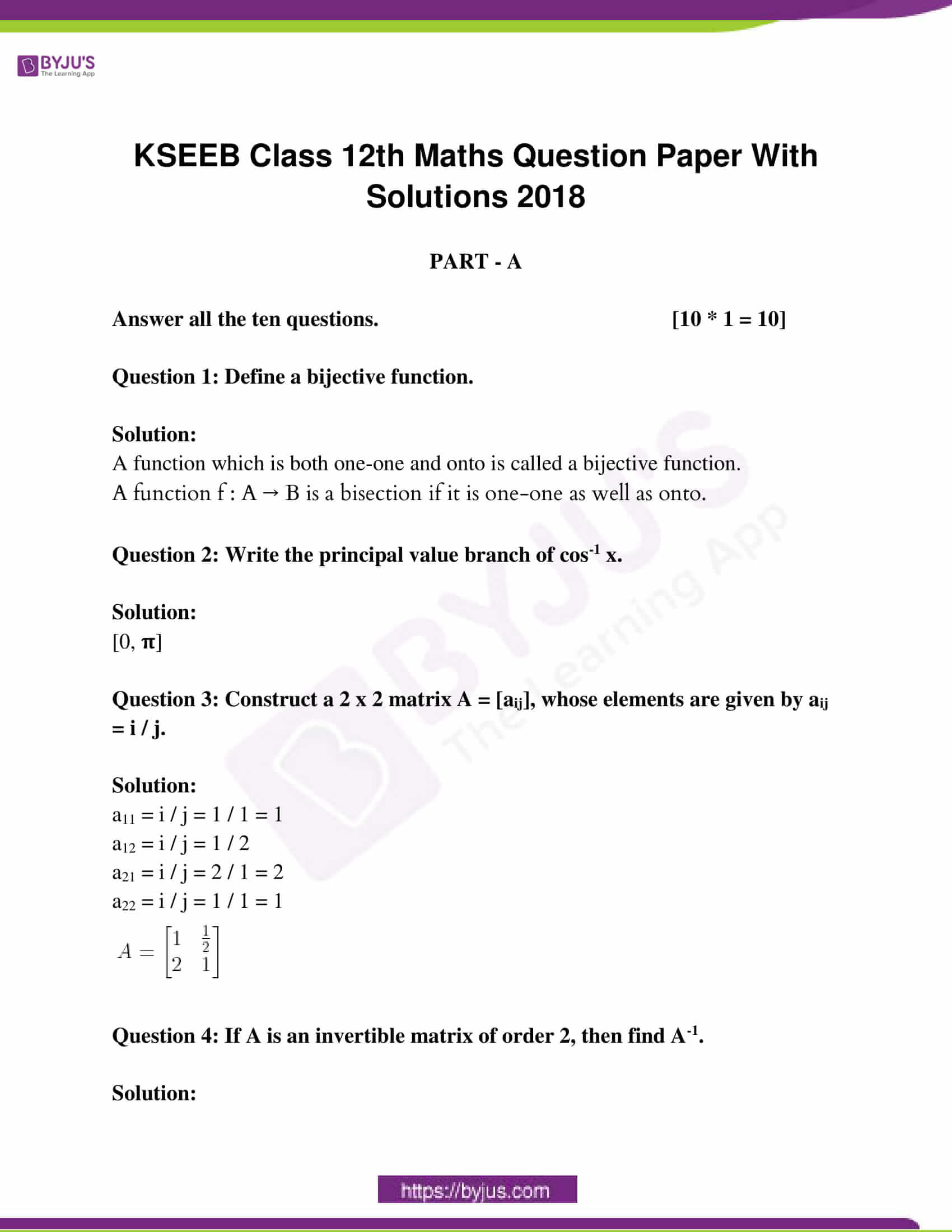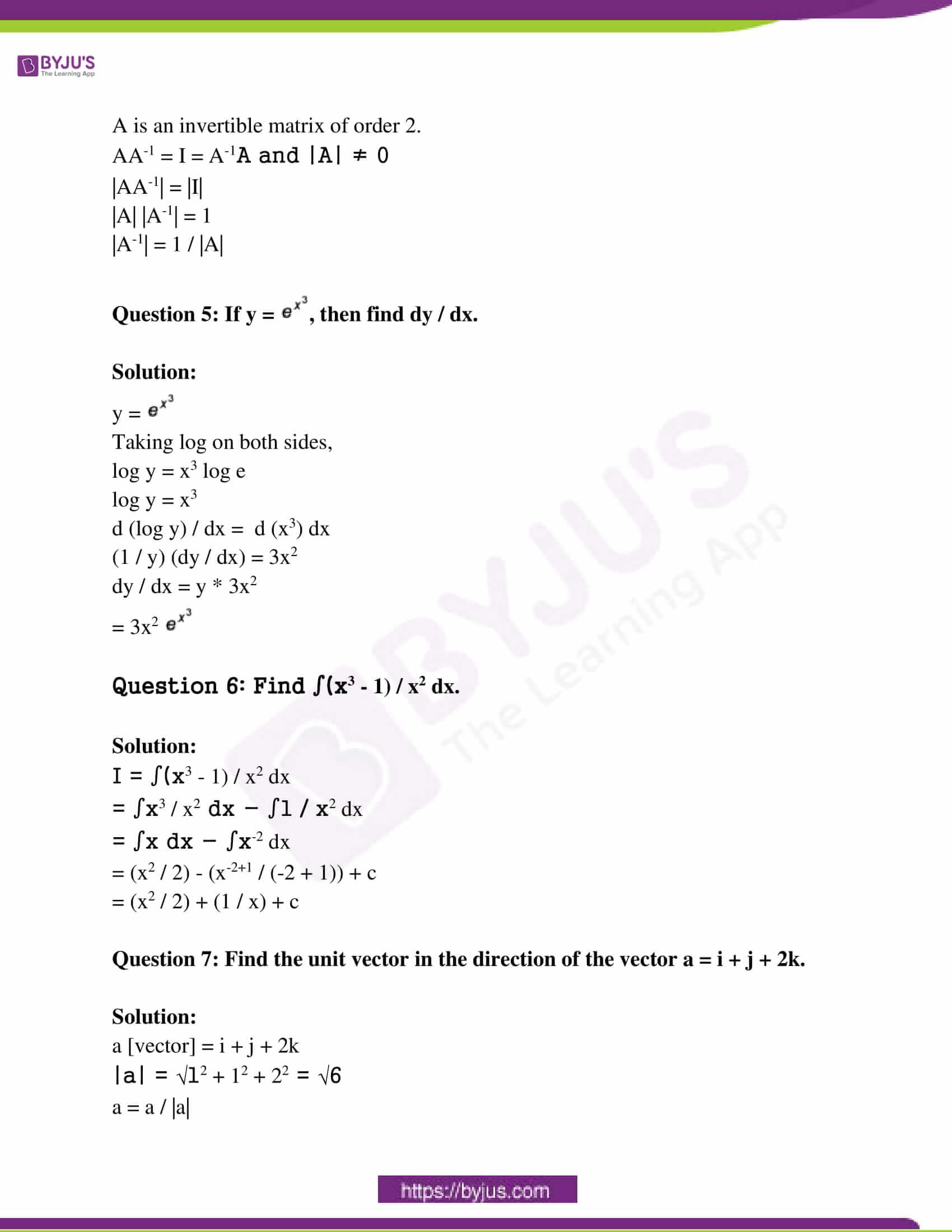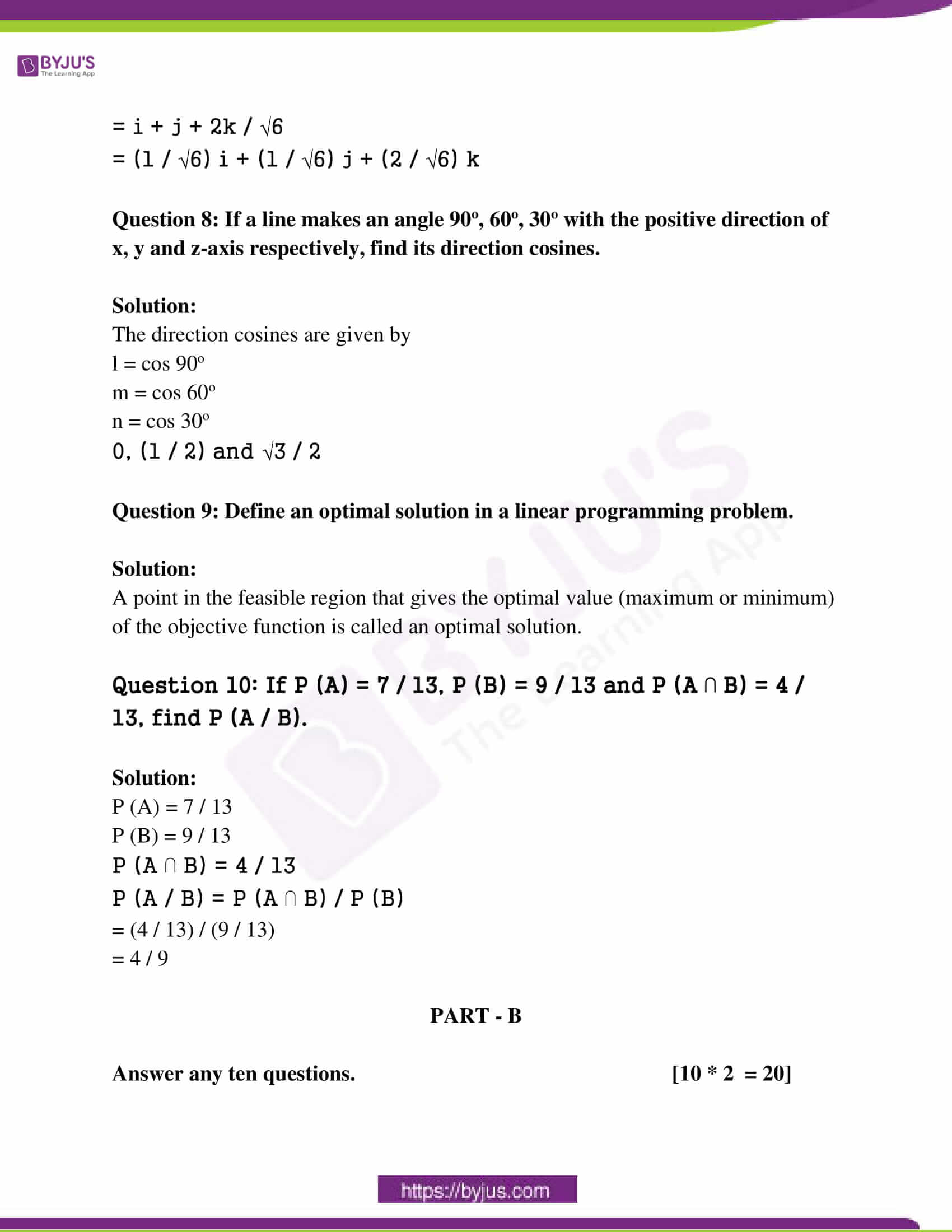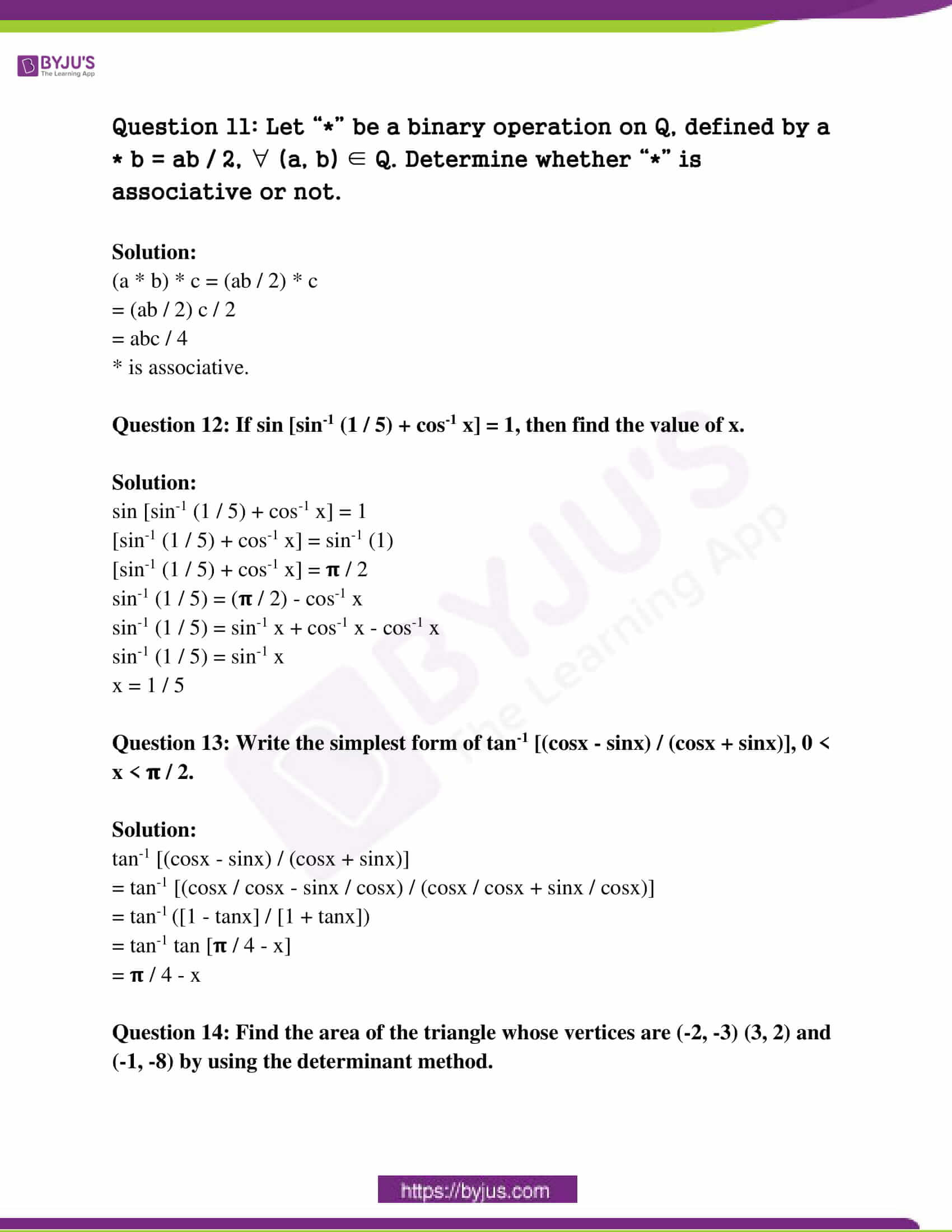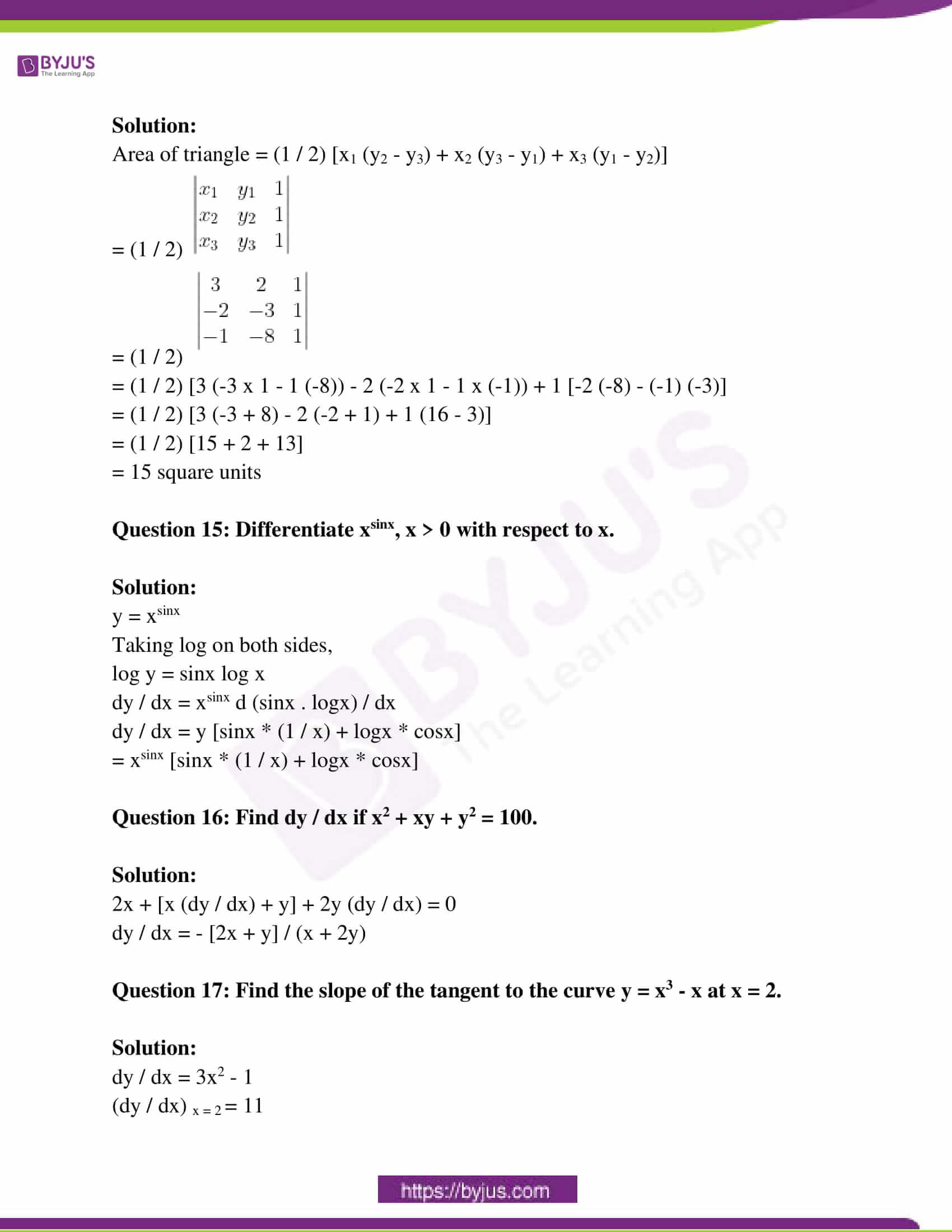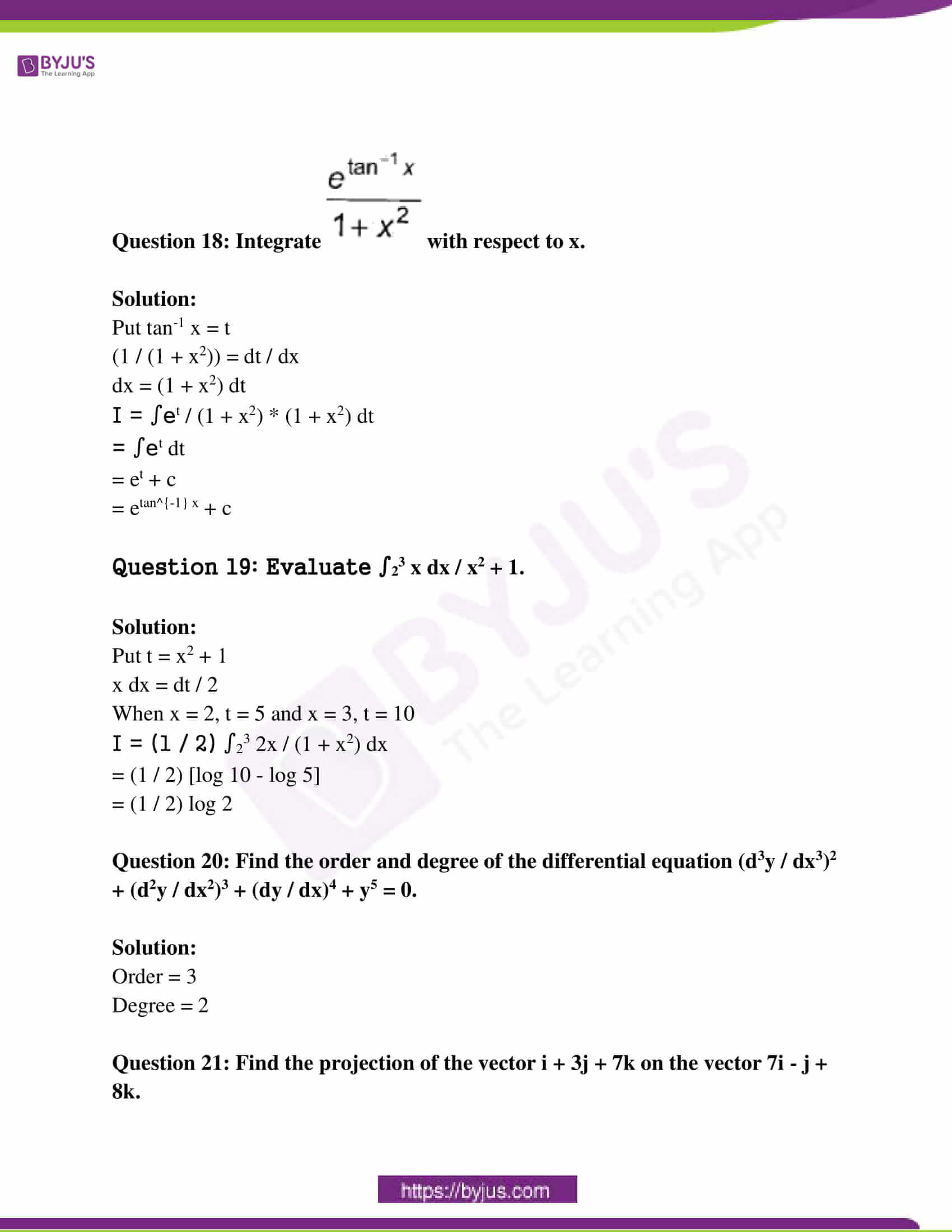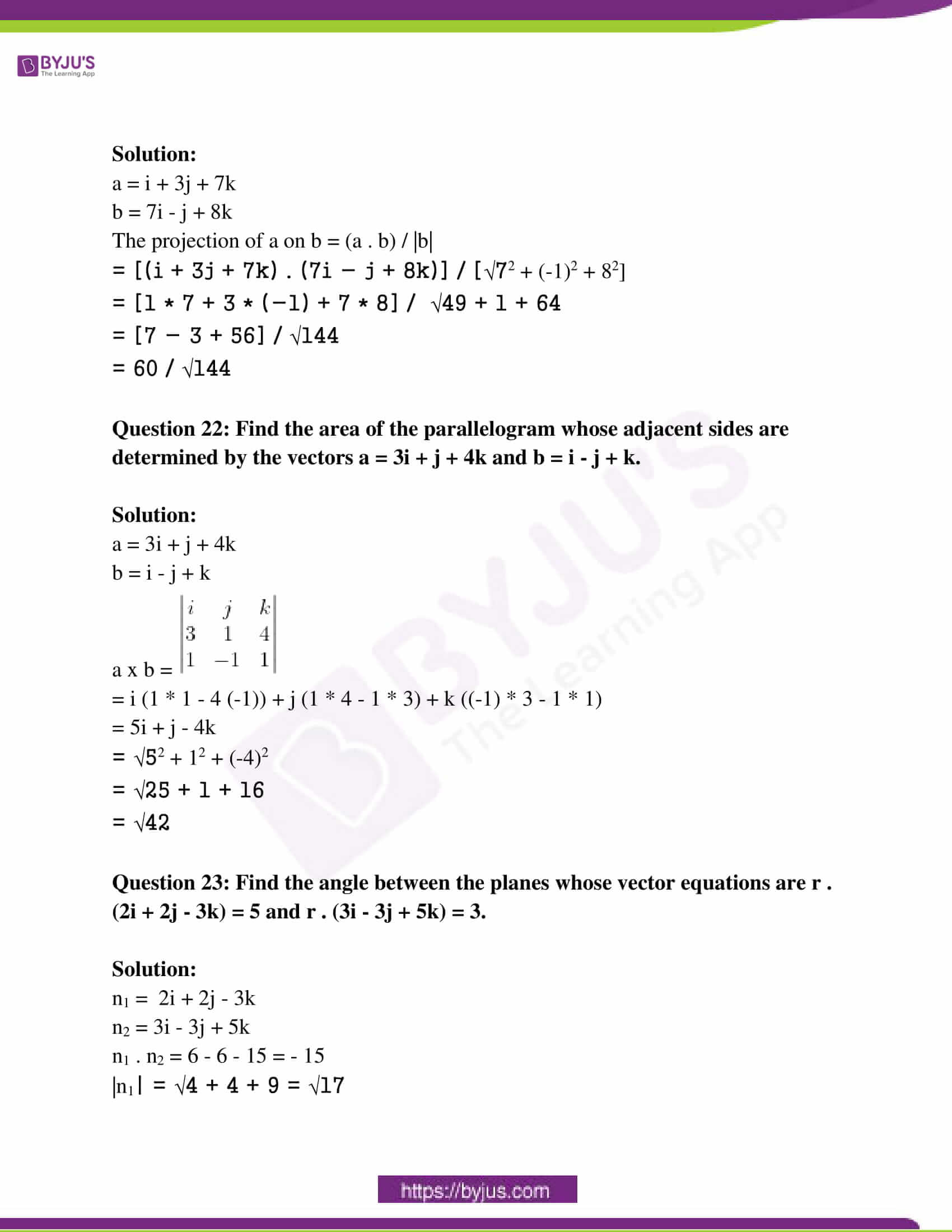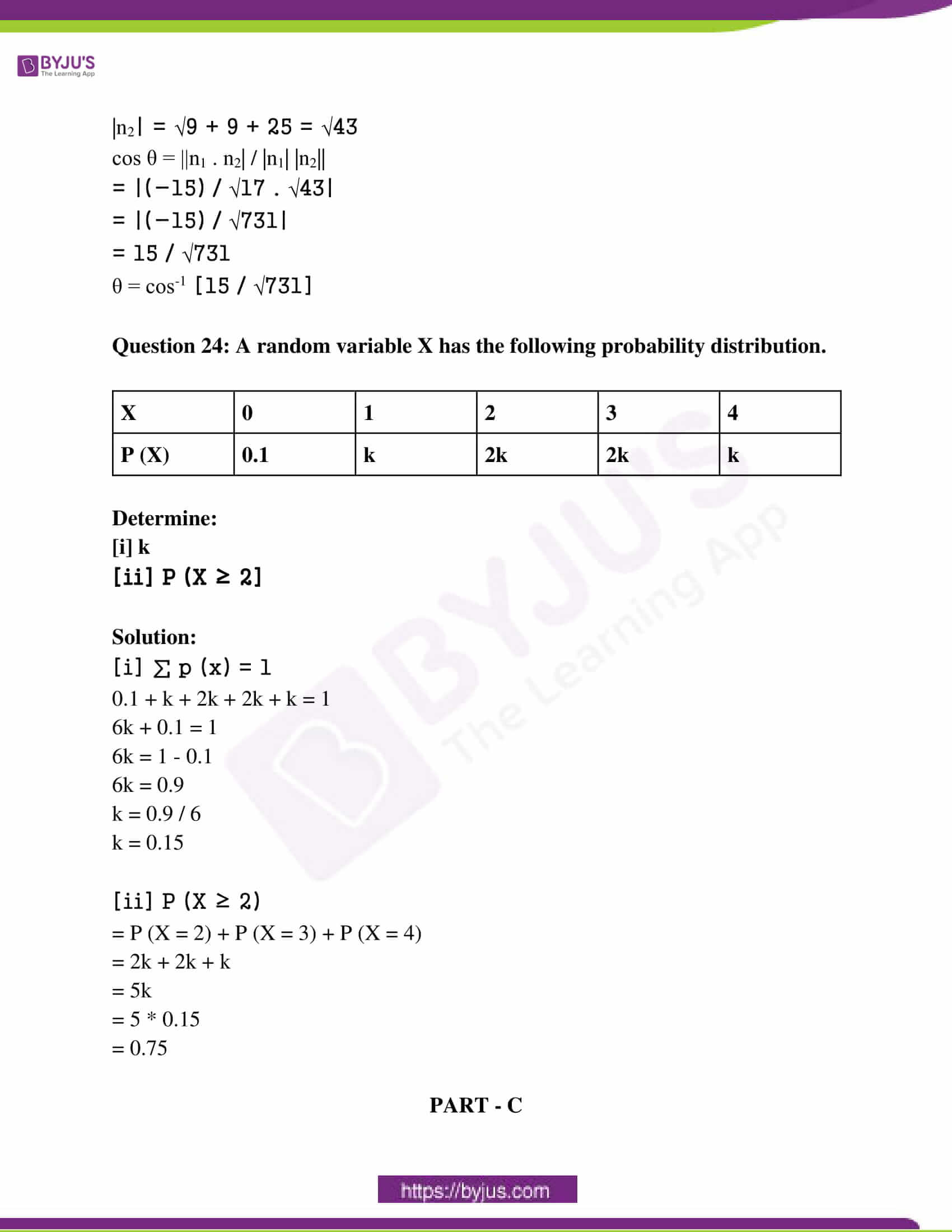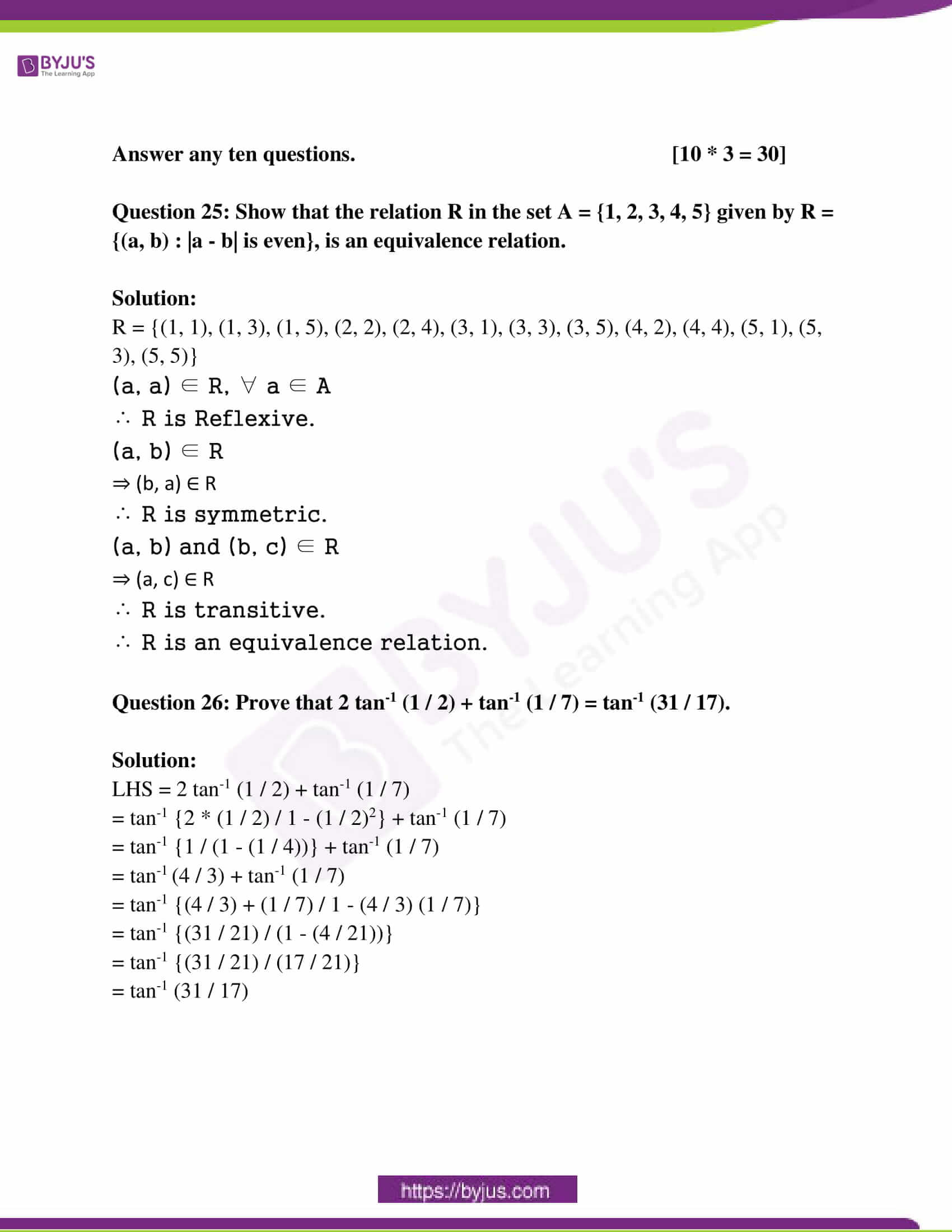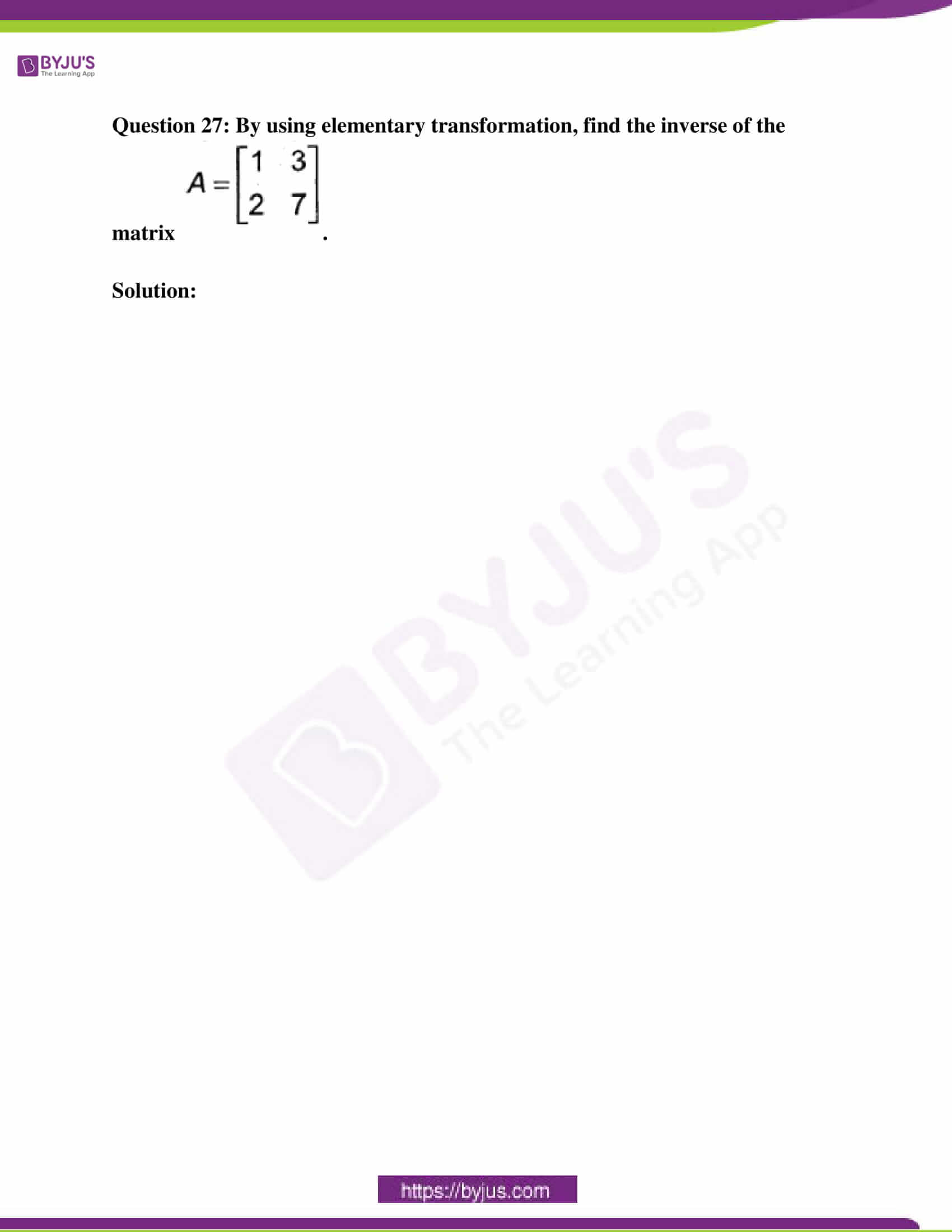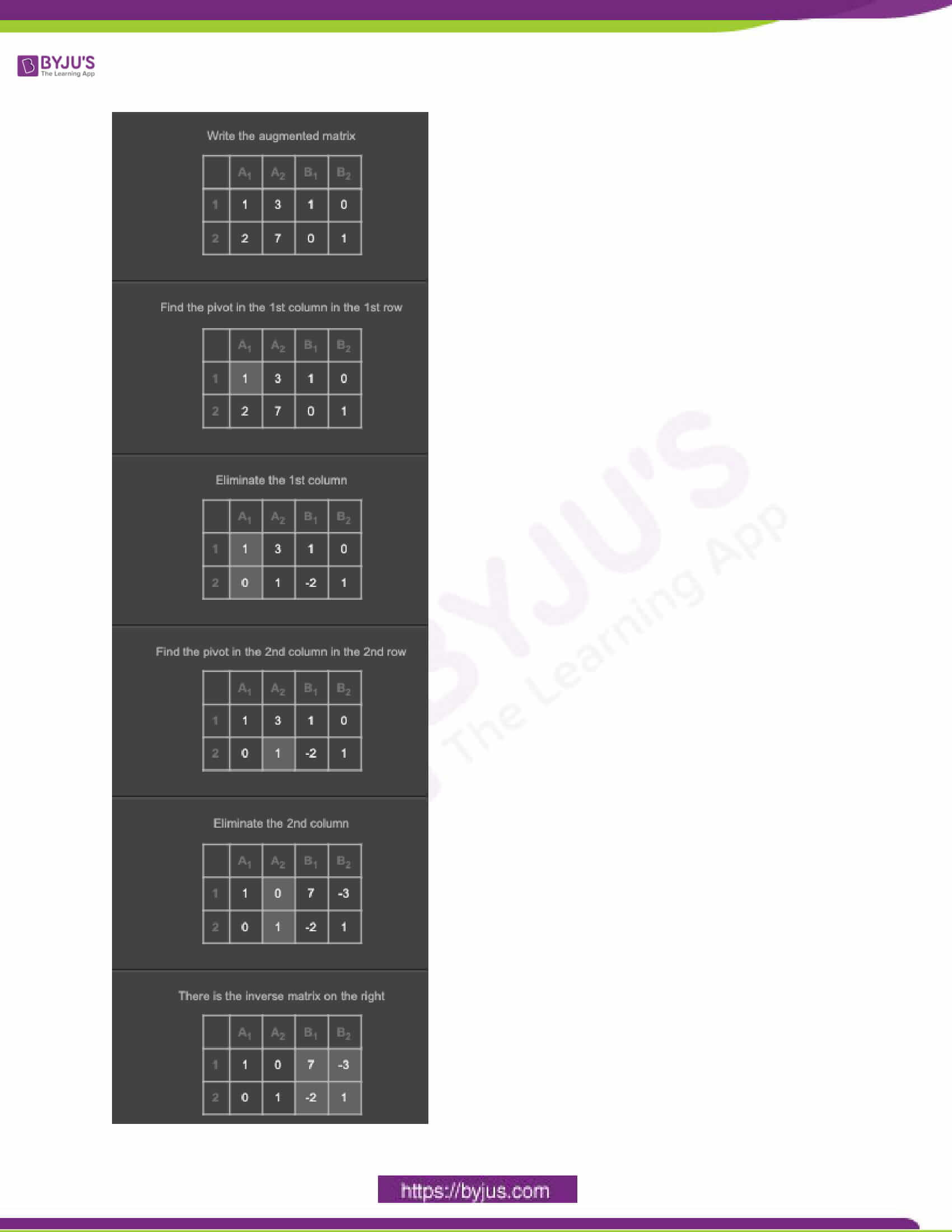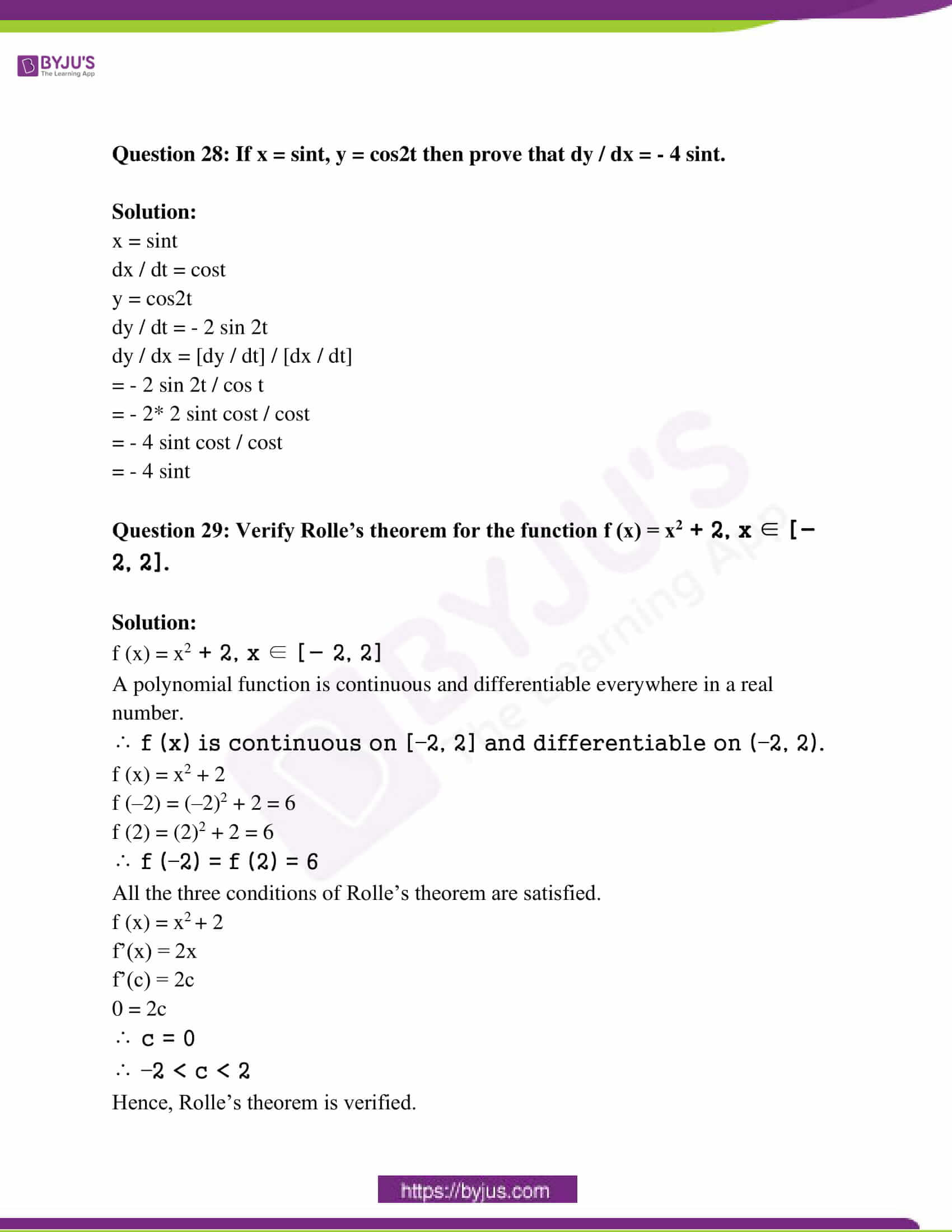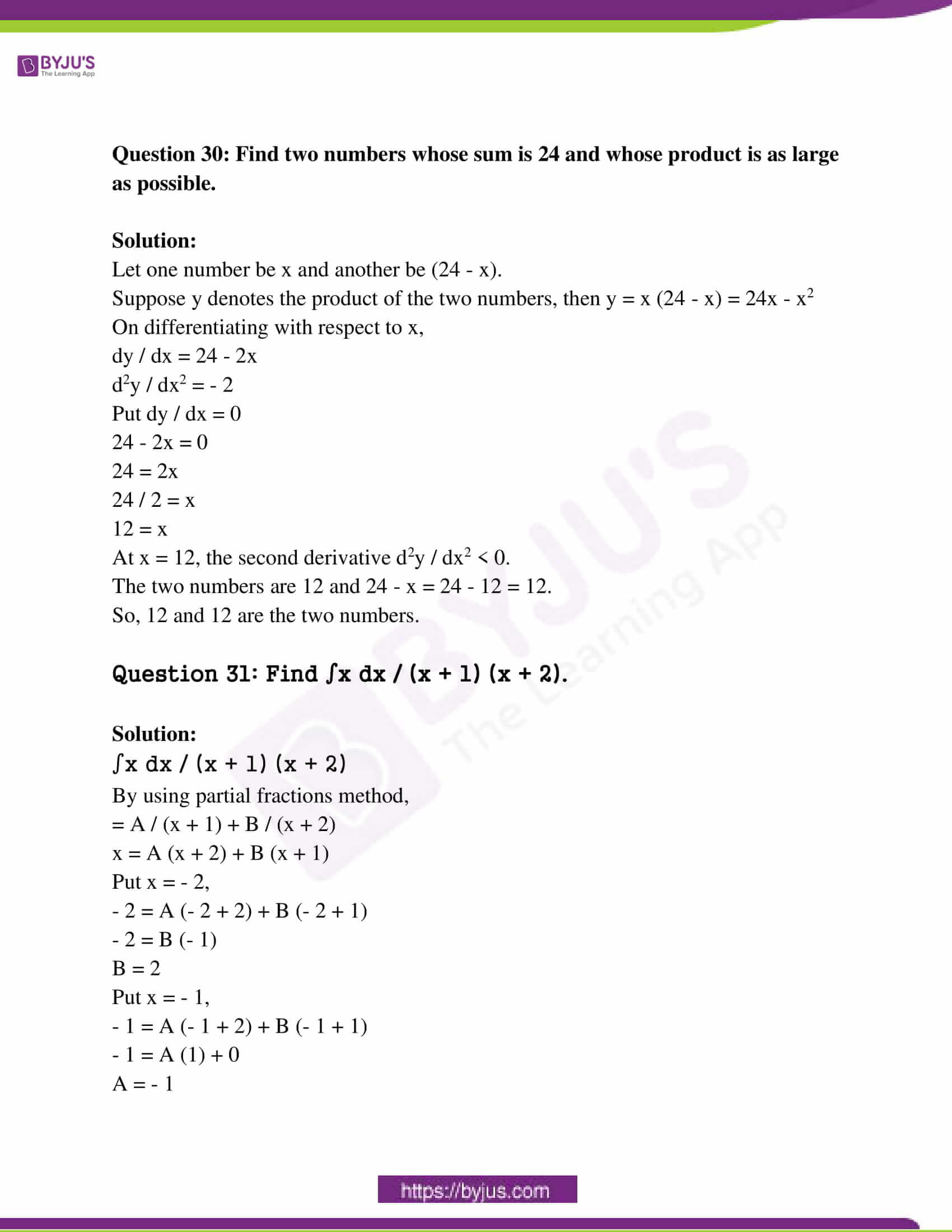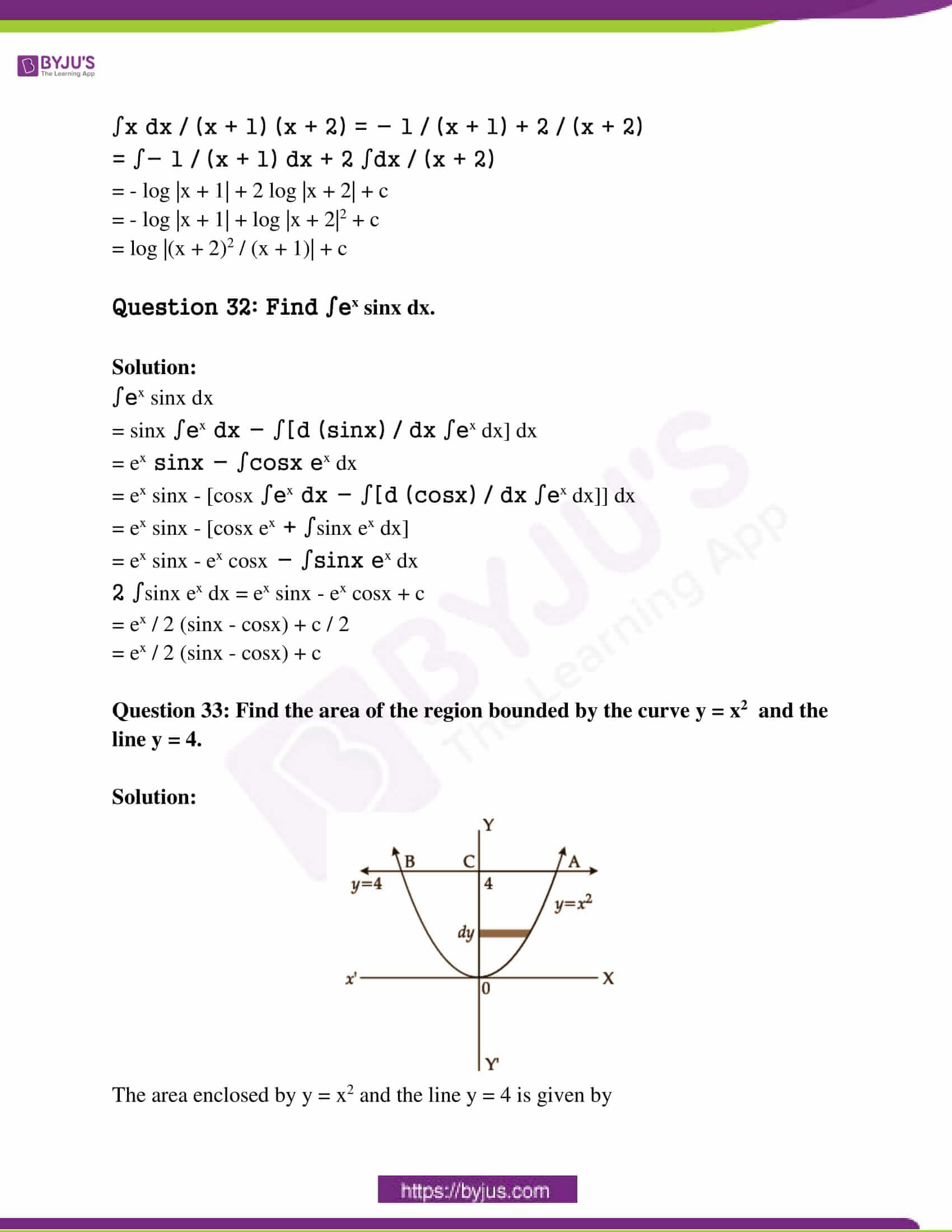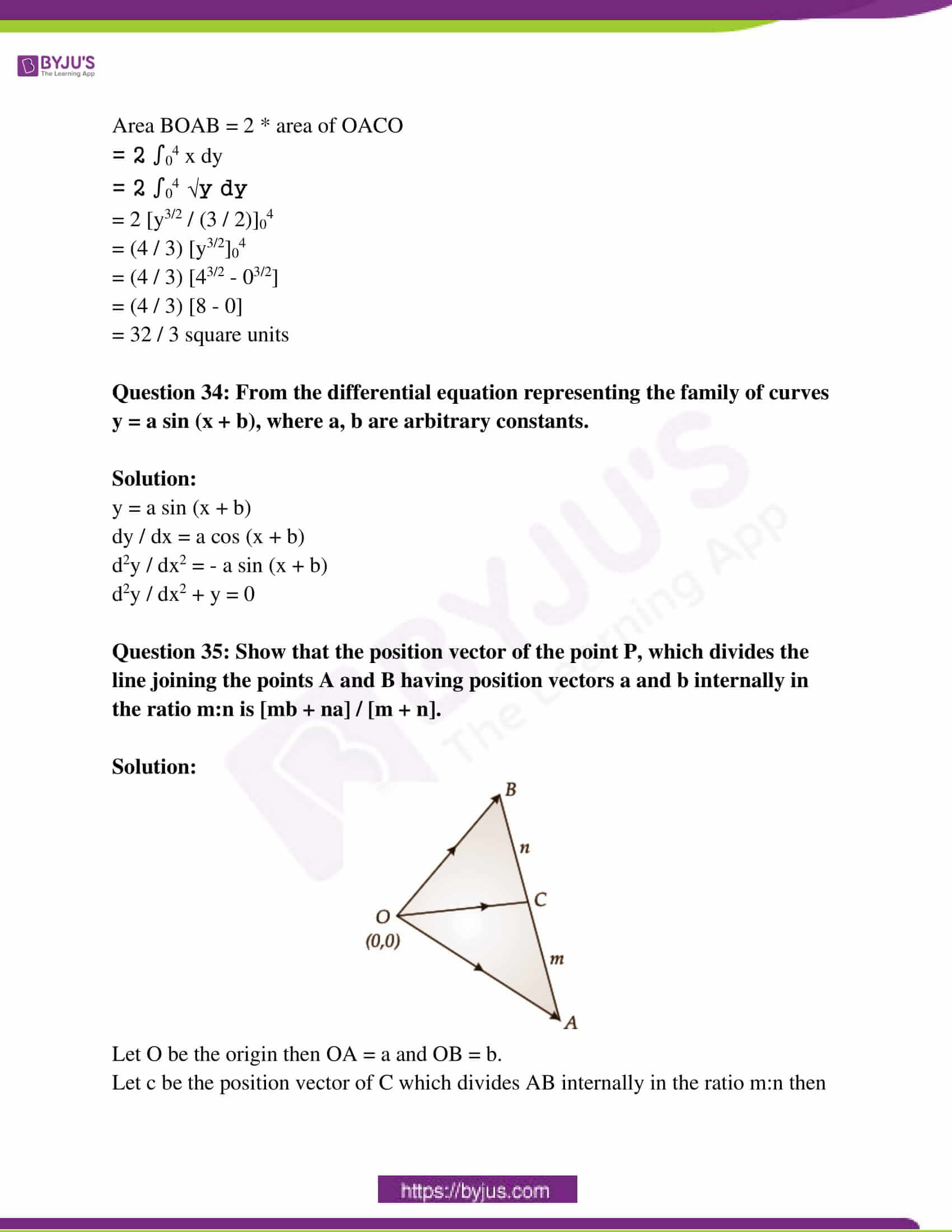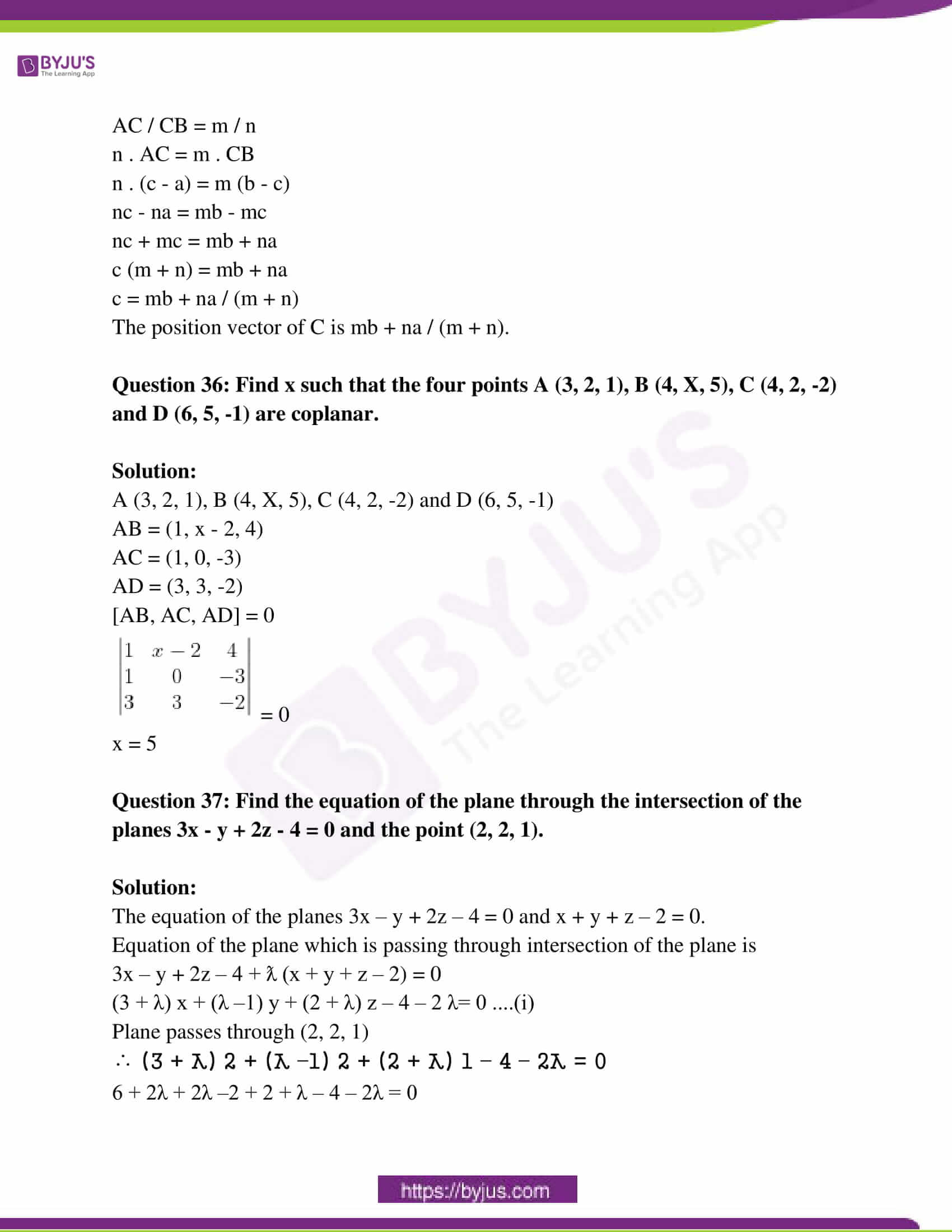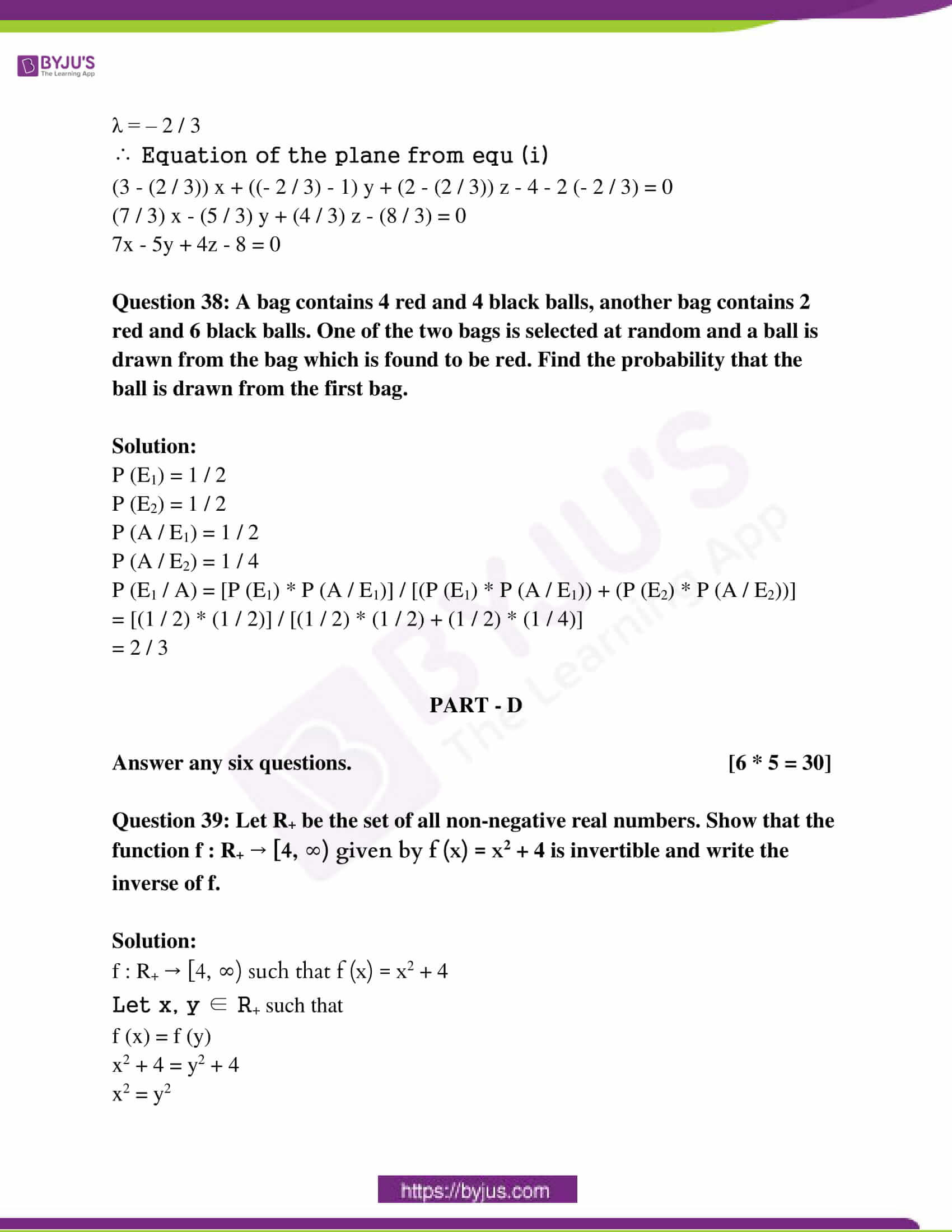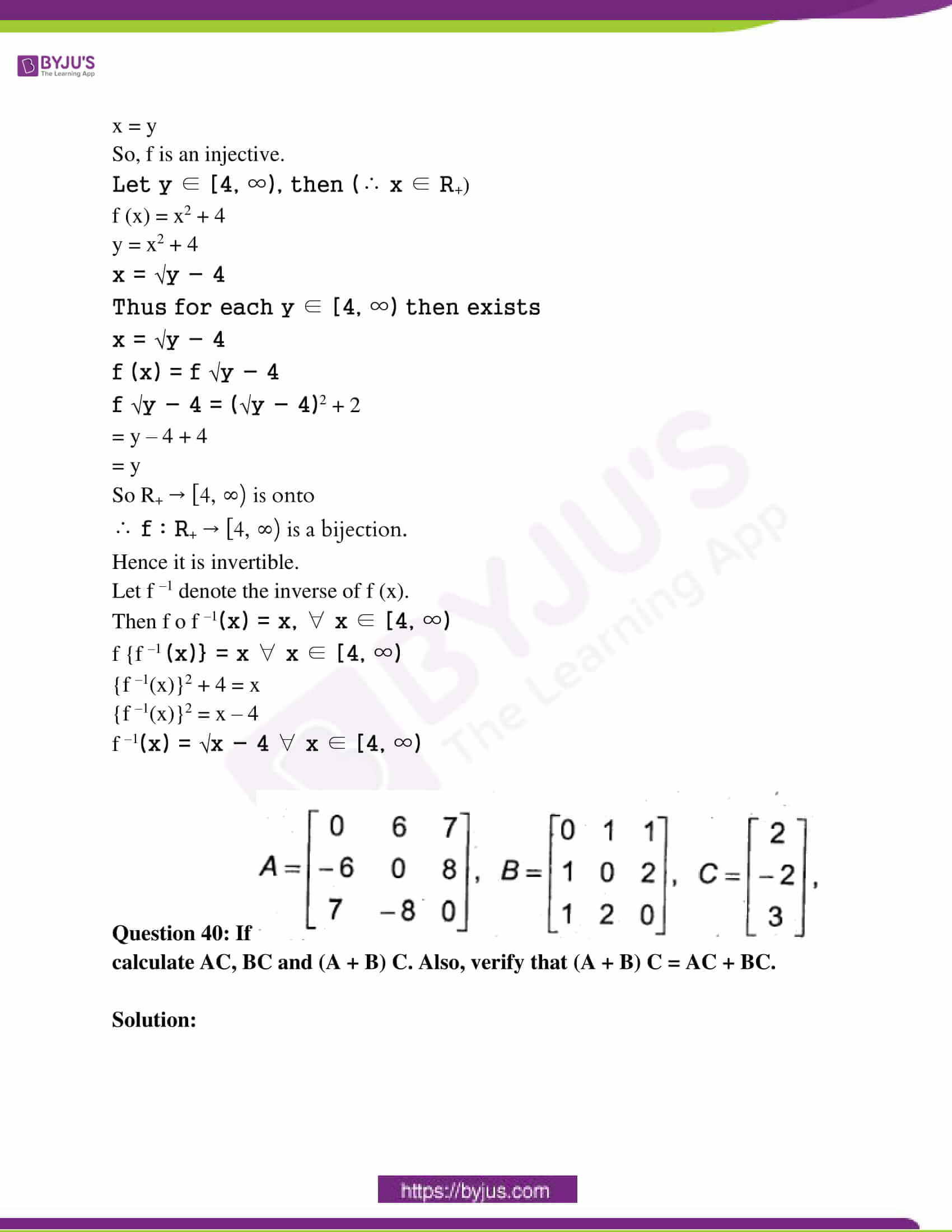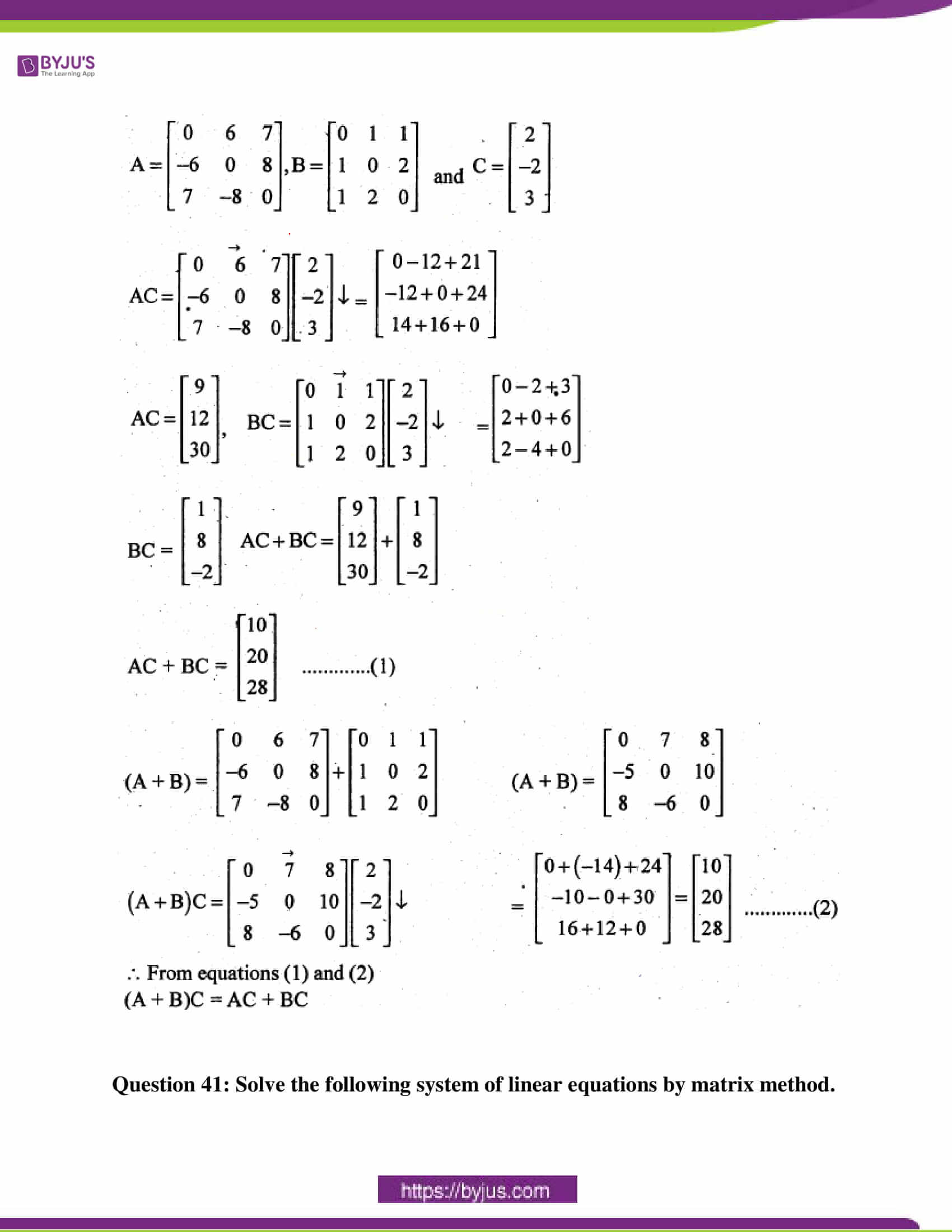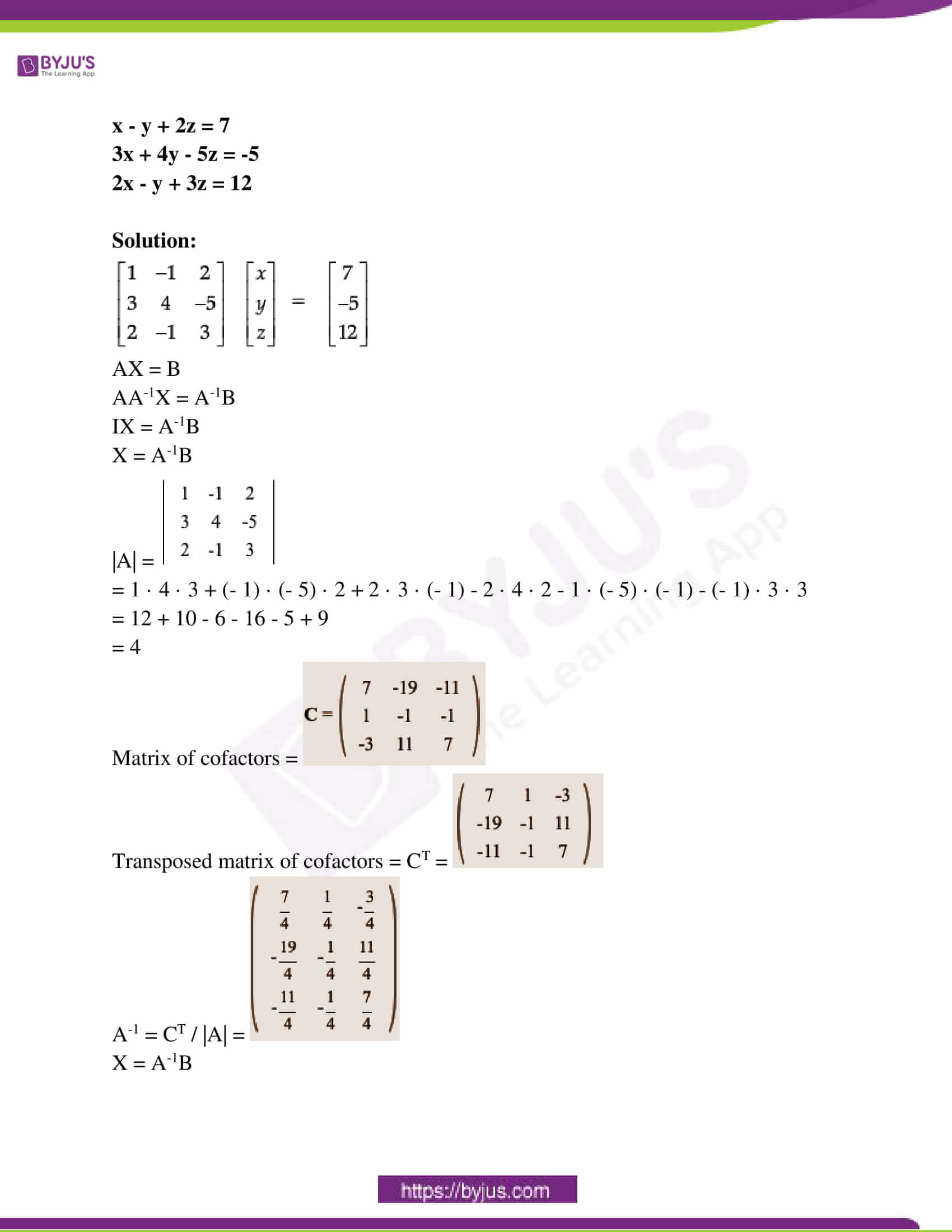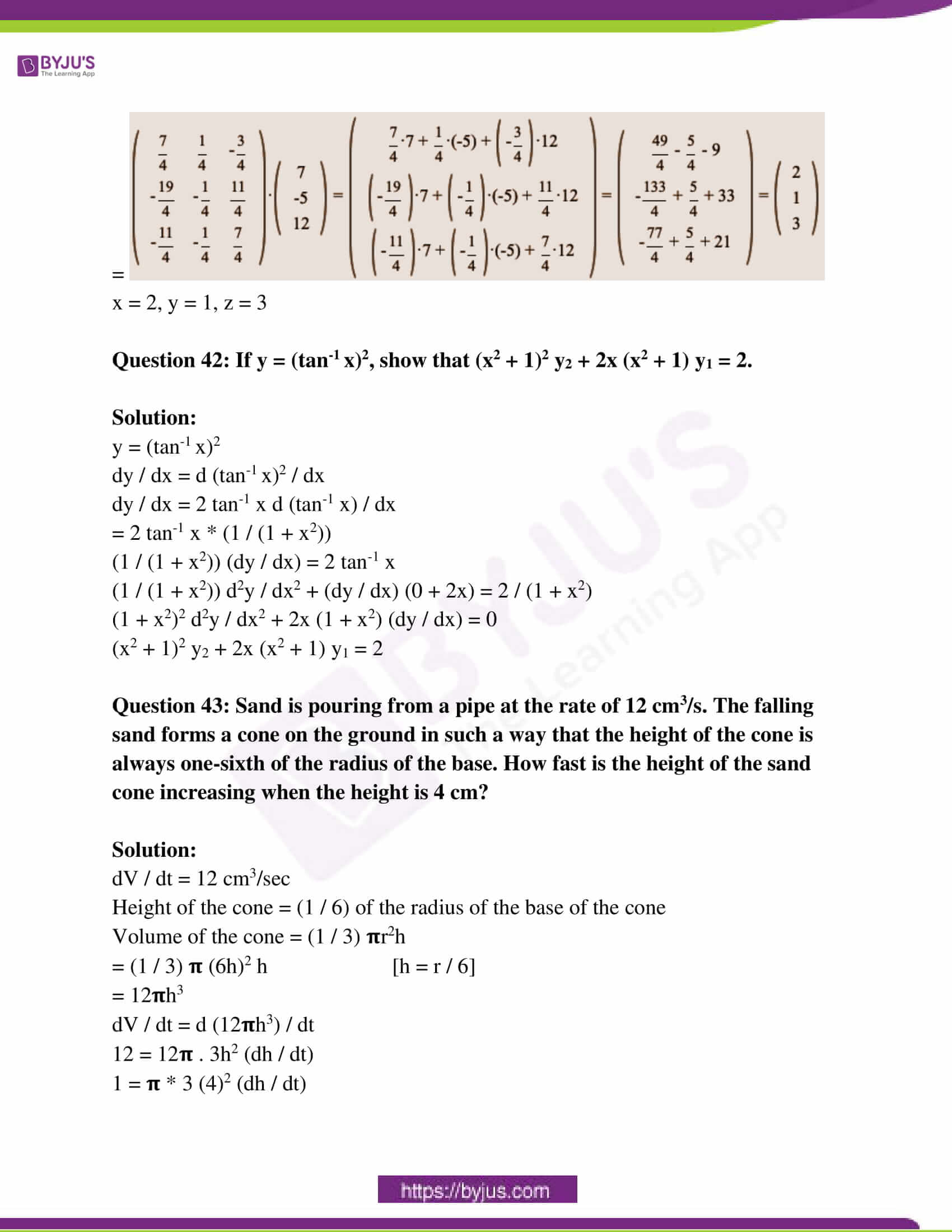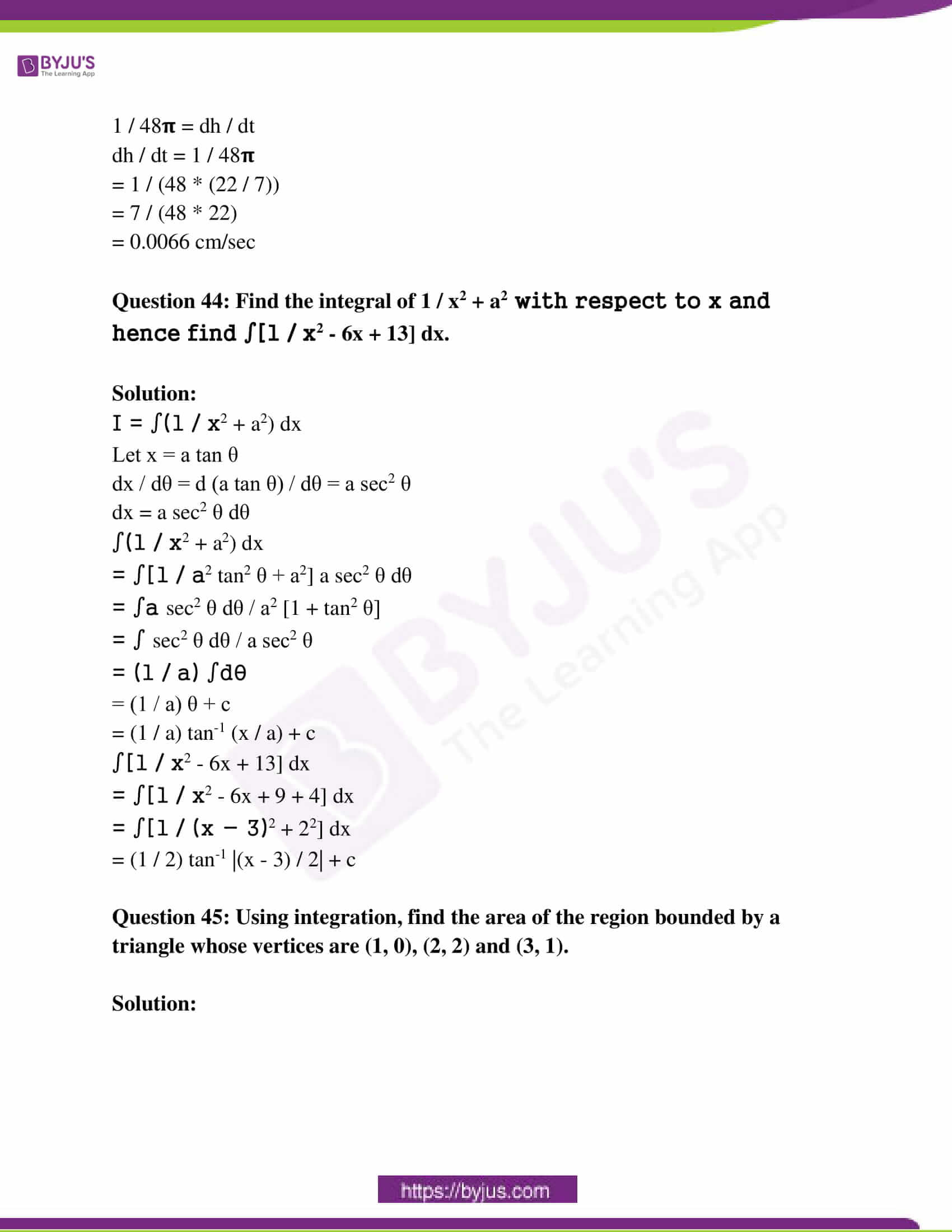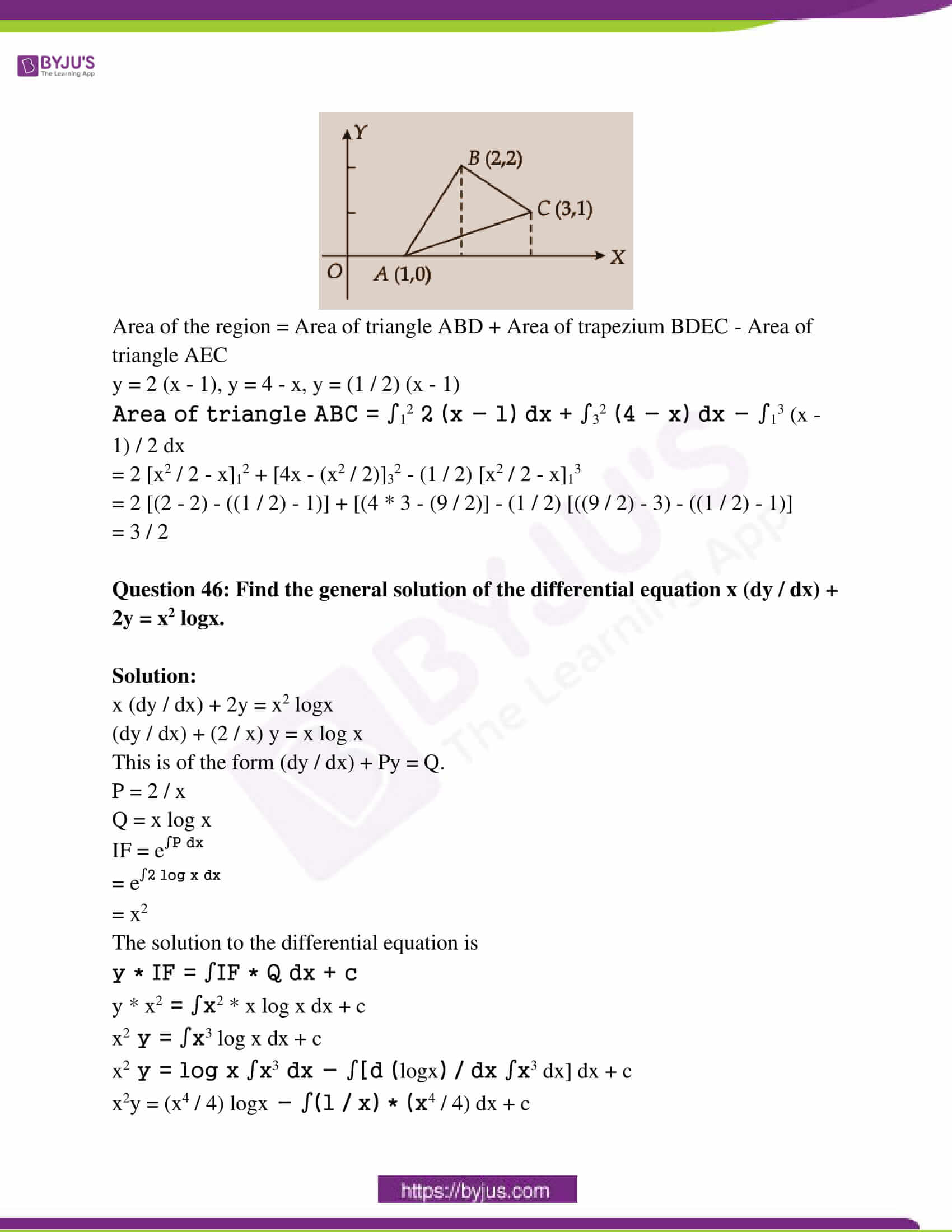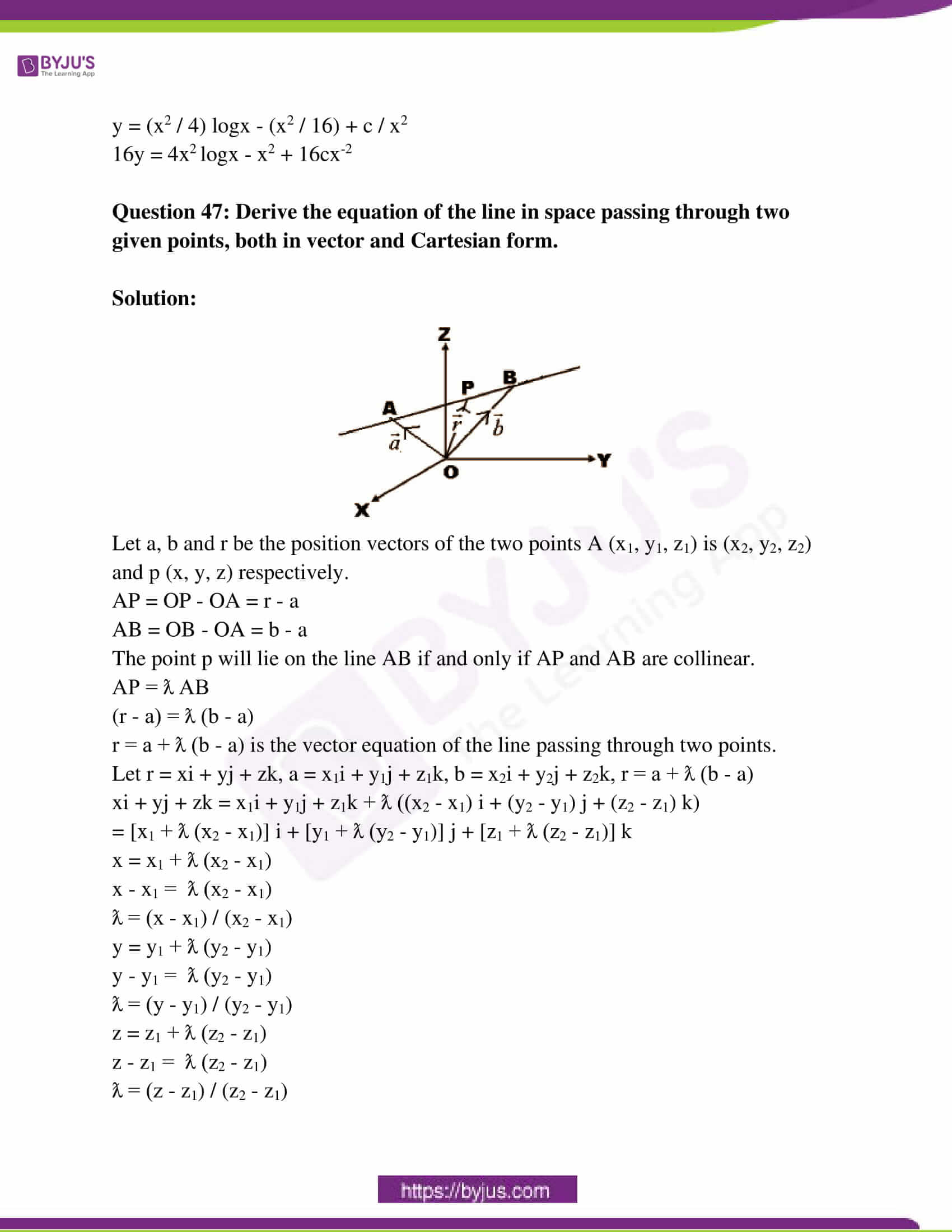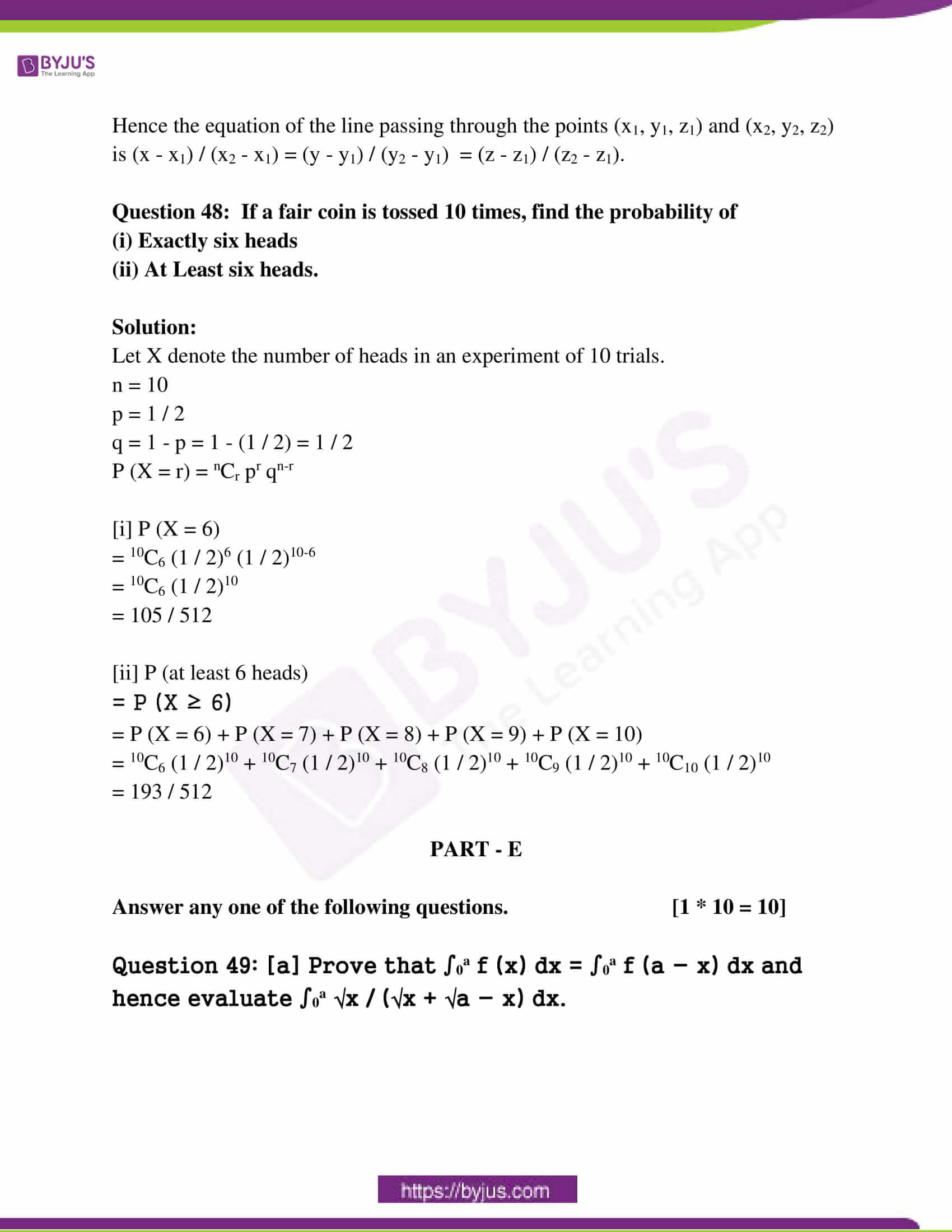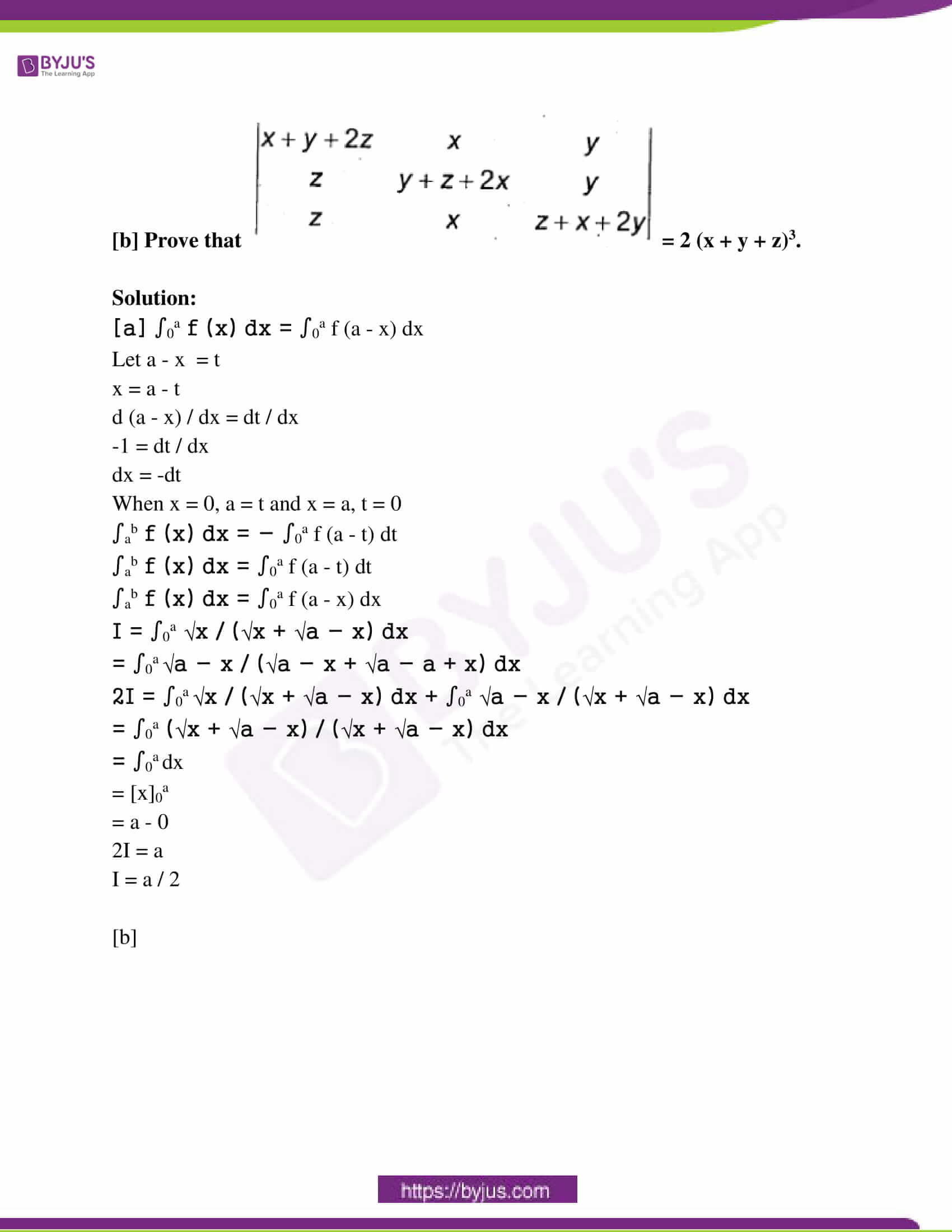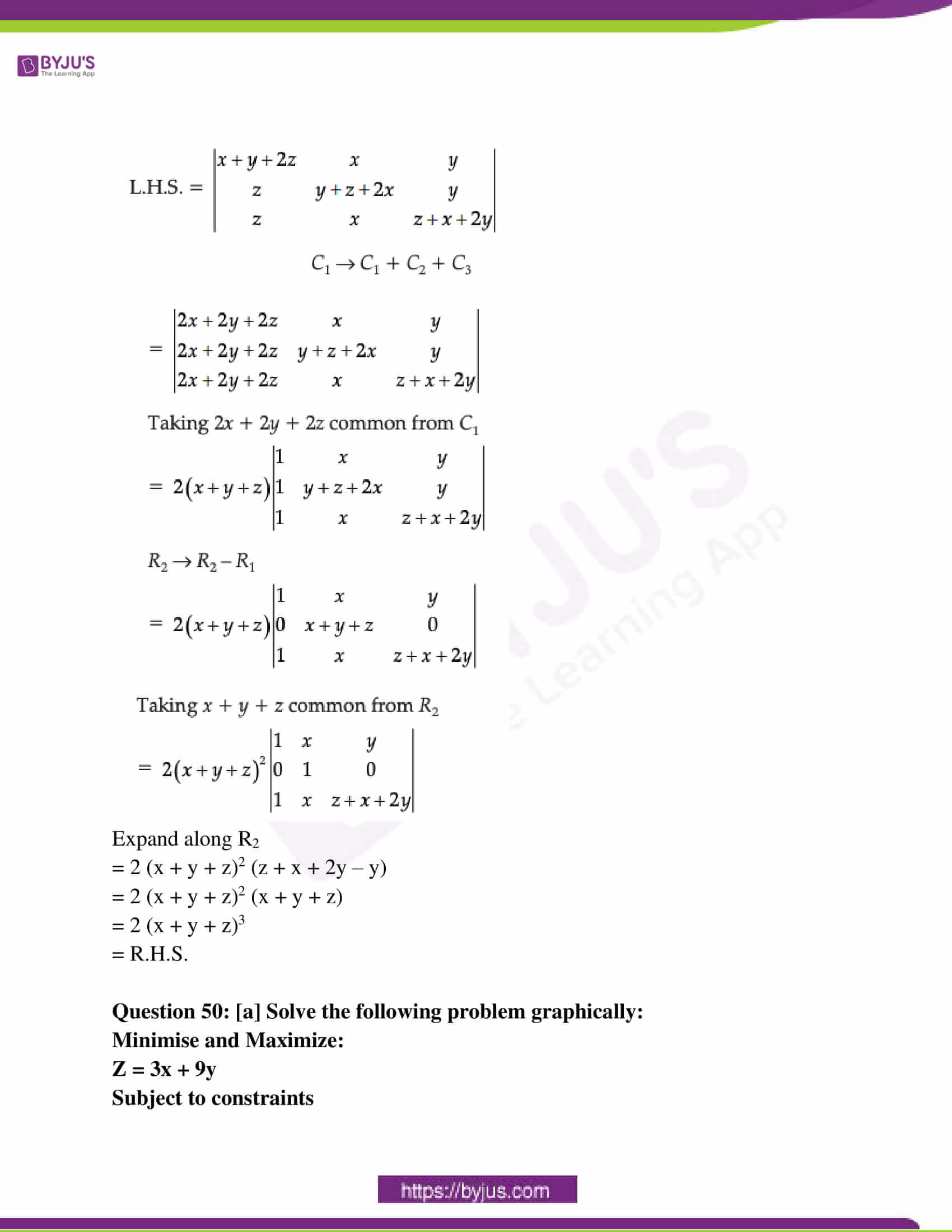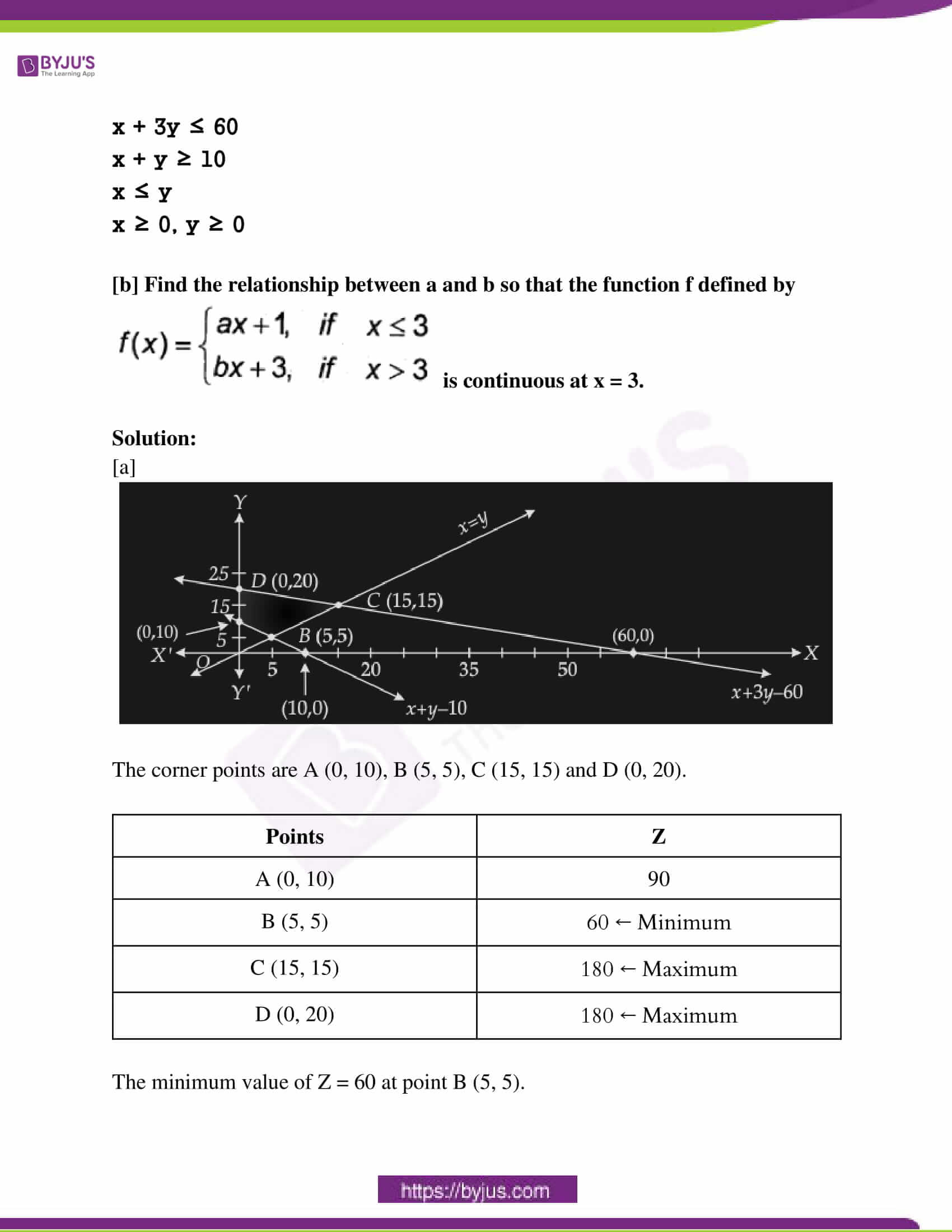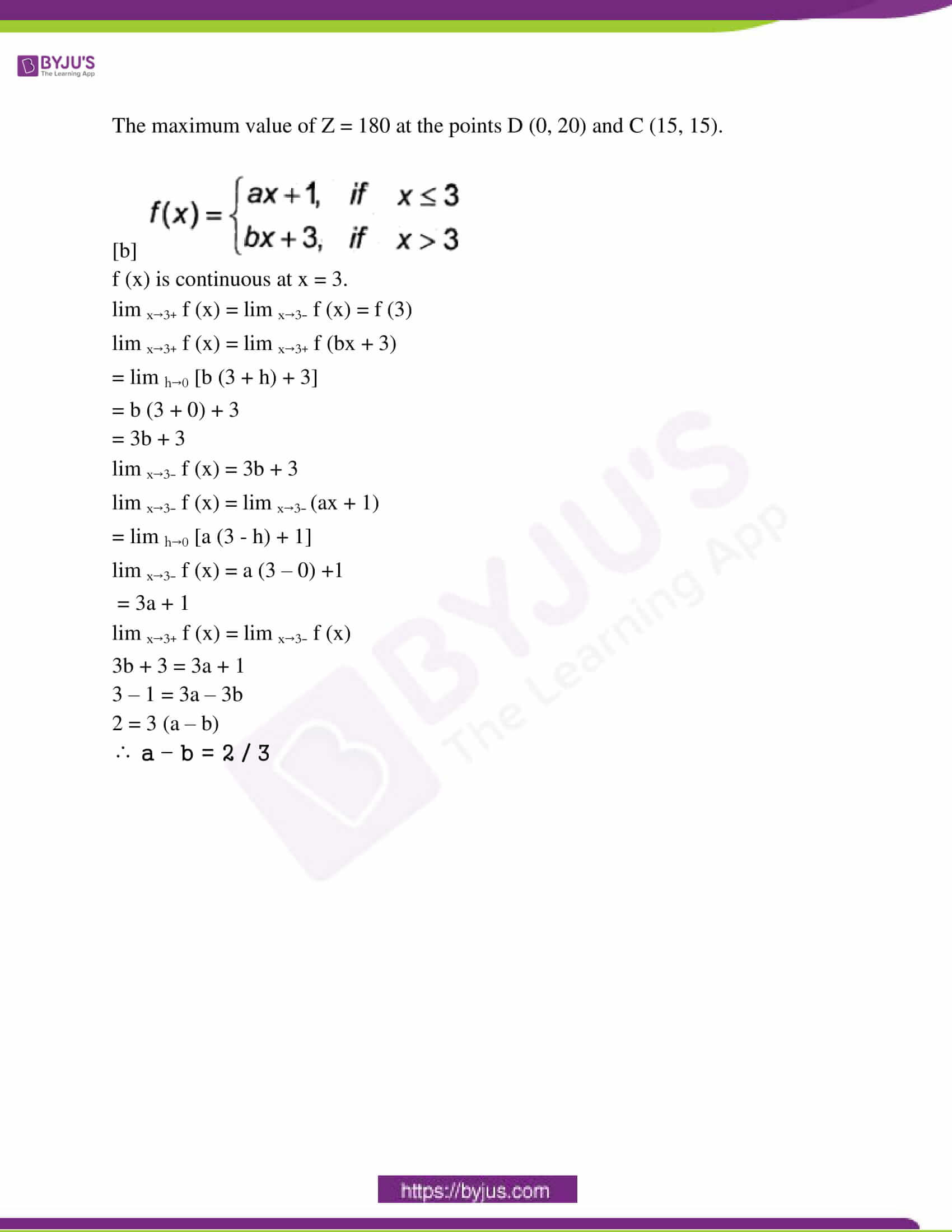PART – A

Answer all the ten questions. [10 * 1 = 10]

Question 1: Define a bijective function.

Solution:

A function which is both one-one and onto is called a bijective function.

A function f : A → B is a bisection if it is one-one as well as onto.

Question 2: Write the principal value branch of cos-1 x.

Solution:

[0, 𝛑]

Question 3: Construct a 2 x 2 matrix A = [aij], whose elements are given by aij = i / j.

Solution:

a11 = i / j = 1 / 1 = 1

a12 = i / j = 1 / 2

a21 = i / j = 2 / 1 = 2

a22 = i / j = 1 / 1 = 1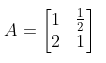Question 4: If A is an invertible matrix of order 2, then find A-1.

Solution:

A is an invertible matrix of order 2.

AA-1 = I = A-1A and |A| ≠ 0

|AA-1| = |I|

|A| |A-1| = 1

|A-1| = 1 / |A|

Question 5: If y =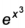, then find dy / dx.

Solution:

y =Taking log on both sides,

log y = x3 log e

log y = x3

d (log y) / dx = d (x3) dx

(1 / y) (dy / dx) = 3x2

dy / dx = y * 3x2

= 3x2Question 6: Find ∫(x3 – 1) / x2 dx.

Solution:

I = ∫(x3 – 1) / x2 dx

= ∫x3 / x2 dx – ∫1 / x2 dx

= ∫x dx – ∫x-2 dx

= (x2 / 2) – (x-2+1 / (-2 + 1)) + c

= (x2 / 2) + (1 / x) + c

Question 7: Find the unit vector in the direction of the vector a = i + j + 2k.

Solution:

a [vector] = i + j + 2k

|a| = √12 + 12 + 22 = √6

a = a / |a|

= i + j + 2k / √6

= (1 / √6) i + (1 / √6) j + (2 / √6) k

Question 8: If a line makes an angle 90o, 60o, 30o with the positive direction of x, y and z-axis respectively, find its direction cosines.

Solution:

The direction cosines are given by

l = cos 90o

m = cos 60o

n = cos 30o

0, (1 / 2) and √3 / 2

Question 9: Define an optimal solution in a linear programming problem.

Solution:

A point in the feasible region that gives the optimal value (maximum or minimum) of the objective function is called an optimal solution.

Question 10: If P (A) = 7 / 13, P (B) = 9 / 13 and P (A ⋂ B) = 4 / 13, find P (A / B).

Solution:

P (A) = 7 / 13

P (B) = 9 / 13

P (A ⋂ B) = 4 / 13

P (A / B) = P (A ⋂ B) / P (B)

= (4 / 13) / (9 / 13)

= 4 / 9

PART – B

Answer any ten questions. [10 * 2 = 20]

Question 11: Let “*” be a binary operation on Q, defined by a * b = ab / 2, ∀ (a, b) ∈ Q. Determine whether “*” is associative or not.

Solution:

(a * b) * c = (ab / 2) * c

= (ab / 2) c / 2

= abc / 4

* is associative.

Question 12: If sin [sin-1 (1 / 5) + cos-1 x] = 1, then find the value of x.

Solution:

sin [sin-1 (1 / 5) + cos-1 x] = 1

[sin-1 (1 / 5) + cos-1 x] = sin-1 (1)

[sin-1 (1 / 5) + cos-1 x] = 𝛑 / 2

sin-1 (1 / 5) = (𝛑 / 2) – cos-1 x

sin-1 (1 / 5) = sin-1 x + cos-1 x – cos-1 x

sin-1 (1 / 5) = sin-1 x

x = 1 / 5

Question 13: Write the simplest form of tan-1 [(cosx – sinx) / (cosx + sinx)], 0 < x < 𝛑 / 2.

Solution:

tan-1 [(cosx – sinx) / (cosx + sinx)]

= tan-1 [(cosx / cosx – sinx / cosx) / (cosx / cosx + sinx / cosx)]

= tan-1 ([1 – tanx] / [1 + tanx])

= tan-1 tan [𝛑 / 4 – x]

= 𝛑 / 4 – x

Question 14: Find the area of the triangle whose vertices are (-2, -3) (3, 2) and (-1, -8) by using the determinant method.

Solution:

Area of triangle = (1 / 2) [x1 (y2 – y3) + x2 (y3 – y1) + x3 (y1 – y2)]

= (1 / 2)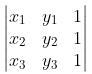= (1 / 2)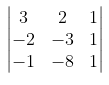= (1 / 2) [3 (-3 x 1 – 1 (-8)) – 2 (-2 x 1 – 1 x (-1)) + 1 [-2 (-8) – (-1) (-3)]

= (1 / 2) [3 (-3 + 8) – 2 (-2 + 1) + 1 (16 – 3)]

= (1 / 2) [15 + 2 + 13]

= 15 square units

Question 15: Differentiate xsinx, x > 0 with respect to x.

Solution:

y = xsinx

Taking log on both sides,

log y = sinx log x

dy / dx = xsinx d (sinx . logx) / dx

dy / dx = y [sinx * (1 / x) + logx * cosx]

= xsinx [sinx * (1 / x) + logx * cosx]

Question 16: Find dy / dx if x2 + xy + y2 = 100.

Solution:

2x + [x (dy / dx) + y] + 2y (dy / dx) = 0

dy / dx = – [2x + y] / (x + 2y)

Question 17: Find the slope of the tangent to the curve y = x3 – x at x = 2.

Solution:

dy / dx = 3x2 – 1

(dy / dx) x = 2 = 11

Question 18: Integrate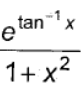with respect to x.

Solution:

Put tan-1 x = t

(1 / (1 + x2)) = dt / dx

dx = (1 + x2) dt

I = ∫et / (1 + x2) * (1 + x2) dt

= ∫et dt

= et + c

= etan^{-1} x + c

Question 19: Evaluate ∫23 x dx / x2 + 1.

Solution:

Put t = x2 + 1

x dx = dt / 2

When x = 2, t = 5 and x = 3, t = 10

I = (1 / 2) ∫23 2x / (1 + x2) dx

= (1 / 2) [log 10 – log 5]

= (1 / 2) log 2

Question 20: Find the order and degree of the differential equation (d3y / dx3)2 + (d2y / dx2)3 + (dy / dx)4 + y5 = 0.

Solution:

Order = 3

Degree = 2

Question 21: Find the projection of the vector i + 3j + 7k on the vector 7i – j + 8k.

Solution:

a = i + 3j + 7k

b = 7i – j + 8k

The projection of a on b = (a . b) / |b|

= [(i + 3j + 7k) . (7i – j + 8k)] / [√72 + (-1)2 + 82]

= [1 * 7 + 3 * (-1) + 7 * 8] / √49 + 1 + 64

= [7 – 3 + 56] / √144

= 60 / √144

Question 22: Find the area of the parallelogram whose adjacent sides are determined by the vectors a = 3i + j + 4k and b = i – j + k.

Solution:

a = 3i + j + 4k

b = i – j + k

a x b =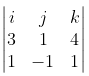= i (1 * 1 – 4 (-1)) + j (1 * 4 – 1 * 3) + k ((-1) * 3 – 1 * 1)

= 5i + j – 4k

= √52 + 12 + (-4)2

= √25 + 1 + 16

= √42

Question 23: Find the angle between the planes whose vector equations are r . (2i + 2j – 3k) = 5 and r . (3i – 3j + 5k) = 3.

Solution:

n1 = 2i + 2j – 3k

n2 = 3i – 3j + 5k

n1 . n2 = 6 – 6 – 15 = – 15

|n1| = √4 + 4 + 9 = √17

|n2| = √9 + 9 + 25 = √43

cos θ = ||n1 . n2| / |n1| |n2||

= |(-15) / √17 . √43|

= |(-15) / √731|

= 15 / √731

θ = cos-1 [15 / √731]

Question 24: A random variable X has the following probability distribution.

 X 0 1 2 3 4 P (X) 0.1 k 2k 2k k

Determine:

[i] k

[ii] P (X ≥ 2]

Solution:

[i] ∑ p (x) = 1

0.1 + k + 2k + 2k + k = 1

6k + 0.1 = 1

6k = 1 – 0.1

6k = 0.9

k = 0.9 / 6

k = 0.15

[ii] P (X ≥ 2)

= P (X = 2) + P (X = 3) + P (X = 4)

= 2k + 2k + k

= 5k

= 5 * 0.15

= 0.75

PART – C

Answer any ten questions. [10 * 3 = 30]

Question 25: Show that the relation R in the set A = {1, 2, 3, 4, 5} given by R = {(a, b) : |a – b| is even}, is an equivalence relation.

Solution:

R = {(1, 1), (1, 3), (1, 5), (2, 2), (2, 4), (3, 1), (3, 3), (3, 5), (4, 2), (4, 4), (5, 1), (5, 3), (5, 5)}

(a, a) ∈ R, ∀ a ∈ A

∴ R is Reflexive.

(a, b) ∈ R

⇒ (b, a) ∈ R

∴ R is symmetric.

(a, b) and (b, c) ∈ R

⇒ (a, c) ∈ R

∴ R is transitive.

∴ R is an equivalence relation.

Question 26: Prove that 2 tan-1 (1 / 2) + tan-1 (1 / 7) = tan-1 (31 / 17).

Solution:

LHS = 2 tan-1 (1 / 2) + tan-1 (1 / 7)

= tan-1 {2 * (1 / 2) / 1 – (1 / 2)2} + tan-1 (1 / 7)

= tan-1 {1 / (1 – (1 / 4))} + tan-1 (1 / 7)

= tan-1 (4 / 3) + tan-1 (1 / 7)

= tan-1 {(4 / 3) + (1 / 7) / 1 – (4 / 3) (1 / 7)}

= tan-1 {(31 / 21) / (1 – (4 / 21))}

= tan-1 {(31 / 21) / (17 / 21)}

= tan-1 (31 / 17)

Question 27: By using elementary transformation, find the inverse of the matrix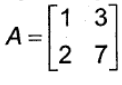.

Solution: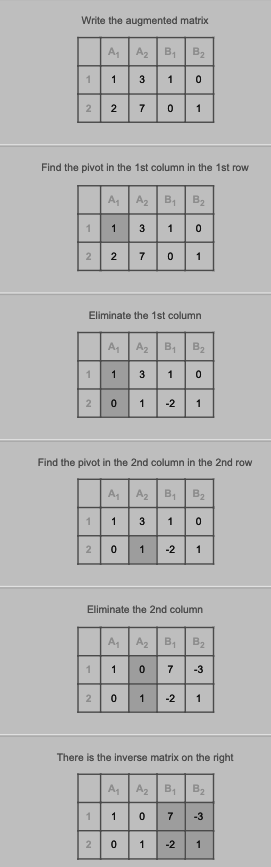Question 28: If x = sint, y = cos2t then prove that dy / dx = – 4 sint.

Solution:

x = sint

dx / dt = cost

y = cos2t

dy / dt = – 2 sin 2t

dy / dx = [dy / dt] / [dx / dt]

= – 2 sin 2t / cos t

= – 2* 2 sint cost / cost

= – 4 sint cost / cost

= – 4 sint

Question 29: Verify Rolle’s theorem for the function f (x) = x2 + 2, x ∈ [- 2, 2].

Solution:

f (x) = x2 + 2, x ∈ [- 2, 2]

A polynomial function is continuous and differentiable everywhere in a real number.

∴ f (x) is continuous on [–2, 2] and differentiable on (–2, 2).

f (x) = x2 + 2

f (–2) = (–2)2 + 2 = 6

f (2) = (2)2 + 2 = 6

∴ f (–2) = f (2) = 6

All the three conditions of Rolle’s theorem are satisfied.

f (x) = x2 + 2

f’(x) = 2x

f’(c) = 2c

0 = 2c

∴ c = 0

∴ –2 < c < 2

Hence, Rolle’s theorem is verified.

Question 30: Find two numbers whose sum is 24 and whose product is as large as possible.

Solution:

Let one number be x and another be (24 – x).

Suppose y denotes the product of the two numbers, then y = x (24 – x) = 24x – x2

On differentiating with respect to x,

dy / dx = 24 – 2x

d2y / dx2 = – 2

Put dy / dx = 0

24 – 2x = 0

24 = 2x

24 / 2 = x

12 = x

At x = 12, the second derivative d2y / dx2 < 0.

The two numbers are 12 and 24 – x = 24 – 12 = 12.

So, 12 and 12 are the two numbers.

Question 31: Find ∫x dx / (x + 1) (x + 2).

Solution:

∫x dx / (x + 1) (x + 2)

By using partial fractions method,

= A / (x + 1) + B / (x + 2)

x = A (x + 2) + B (x + 1)

Put x = – 2,

– 2 = A (- 2 + 2) + B (- 2 + 1)

– 2 = B (- 1)

B = 2

Put x = – 1,

– 1 = A (- 1 + 2) + B (- 1 + 1)

– 1 = A (1) + 0

A = – 1

∫x dx / (x + 1) (x + 2) = – 1 / (x + 1) + 2 / (x + 2)

= ∫- 1 / (x + 1) dx + 2 ∫dx / (x + 2)

= – log |x + 1| + 2 log |x + 2| + c

= – log |x + 1| + log |x + 2|2 + c

= log |(x + 2)2 / (x + 1)| + c

Question 32: Find ∫ex sinx dx.

Solution:

∫ex sinx dx

= sinx ∫ex dx – ∫[d (sinx) / dx ∫ex dx] dx

= ex sinx – ∫cosx ex dx

= ex sinx – [cosx ∫ex dx – ∫[d (cosx) / dx ∫ex dx]] dx

= ex sinx – [cosx ex + ∫sinx ex dx]

= ex sinx – ex cosx – ∫sinx ex dx

2 ∫sinx ex dx = ex sinx – ex cosx + c

= ex / 2 (sinx – cosx) + c / 2

= ex / 2 (sinx – cosx) + c

Question 33: Find the area of the region bounded by the curve y = x2 and the line y = 4.

Solution: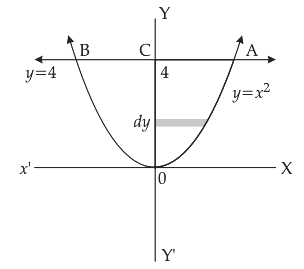The area enclosed by y = x2 and the line y = 4 is given by

Area BOAB = 2 * area of OACO

= 2 ∫04 x dy

= 2 ∫04 √y dy

= 2 [y3/2 / (3 / 2)]04

= (4 / 3) [y3/2]04

= (4 / 3) [43/2 – 03/2]

= (4 / 3) [8 – 0]

= 32 / 3 square units

Question 34: From the differential equation representing the family of curves y = a sin (x + b), where a, b are arbitrary constants.

Solution:

y = a sin (x + b)

dy / dx = a cos (x + b)

d2y / dx2 = – a sin (x + b)

d2y / dx2 + y = 0

Question 35: Show that the position vector of the point P, which divides the line joining the points A and B having position vectors a and b internally in the ratio m:n is [mb + na] / [m + n].

Solution: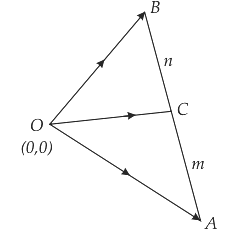Let O be the origin then OA = a and OB = b.

Let c be the position vector of C which divides AB internally in the ratio m:n then

AC / CB = m / n

n . AC = m . CB

n . (c – a) = m (b – c)

nc – na = mb – mc

nc + mc = mb + na

c (m + n) = mb + na

c = mb + na / (m + n)

The position vector of C is mb + na / (m + n).

Question 36: Find x such that the four points A (3, 2, 1), B (4, X, 5), C (4, 2, -2) and D (6, 5, -1) are coplanar.

Solution:

A (3, 2, 1), B (4, X, 5), C (4, 2, -2) and D (6, 5, -1)

AB = (1, x – 2, 4)

AC = (1, 0, -3)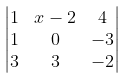= 0

x = 5

Question 37: Find the equation of the plane through the intersection of the planes 3x – y + 2z – 4 = 0 and the point (2, 2, 1).

Solution:

The equation of the planes 3x – y + 2z – 4 = 0 and x + y + z – 2 = 0.

Equation of the plane which is passing through intersection of the plane is

3x – y + 2z – 4 + ƛ (x + y + z – 2) = 0

(3 + λ) x + (λ –1) y + (2 + λ) z – 4 – 2 λ= 0 ….(i)

Plane passes through (2, 2, 1)

∴ (3 + λ) 2 + (λ –1) 2 + (2 + λ) 1 – 4 – 2λ = 0

6 + 2λ + 2λ –2 + 2 + λ – 4 – 2λ = 0

λ = – 2 / 3

∴ Equation of the plane from equ (i)

(3 – (2 / 3)) x + ((- 2 / 3) – 1) y + (2 – (2 / 3)) z – 4 – 2 (- 2 / 3) = 0

(7 / 3) x – (5 / 3) y + (4 / 3) z – (8 / 3) = 0

7x – 5y + 4z – 8 = 0

Question 38: A bag contains 4 red and 4 black balls, another bag contains 2 red and 6 black balls. One of the two bags is selected at random and a ball is drawn from the bag which is found to be red. Find the probability that the ball is drawn from the first bag.

Solution:

P (E1) = 1 / 2

P (E2) = 1 / 2

P (A / E1) = 1 / 2

P (A / E2) = 1 / 4

P (E1 / A) = [P (E1) * P (A / E1)] / [(P (E1) * P (A / E1)) + (P (E2) * P (A / E2))]

= [(1 / 2) * (1 / 2)] / [(1 / 2) * (1 / 2) + (1 / 2) * (1 / 4)]

= 2 / 3

PART – D

Answer any six questions. [6 * 5 = 30]

Question 39: Let R+ be the set of all non-negative real numbers. Show that the function f : R+ → [4, ∞) given by f (x) = x2 + 4 is invertible and write the inverse of f.

Solution:

f : R+ → [4, ∞) such that f (x) = x2 + 4

Let x, y ∈ R+ such that

f (x) = f (y)

x2 + 4 = y2 + 4

x2 = y2

x = y

So, f is an injective.

Let y ∈ [4, ∞), then (∴ x ∈ R+)

f (x) = x2 + 4

y = x2 + 4

x = √y − 4

Thus for each y ∈ [4, ∞) then exists

x = √y − 4

f (x) = f √y − 4

f √y − 4 = (√y − 4)2 + 2

= y – 4 + 4

= y

So R+ → [4, ∞) is onto

∴ f : R+ → [4, ∞) is a bijection.

Hence it is invertible.

Let f –1 denote the inverse of f (x).

Then f o f –1(x) = x, ∀ x ∈ [4, ∞)

f {f –1 (x)} = x ∀ x ∈ [4, ∞)

{f –1(x)}2 + 4 = x

{f –1(x)}2 = x – 4

f –1(x) = √x − 4 ∀ x ∈ [4, ∞)

Question 40: If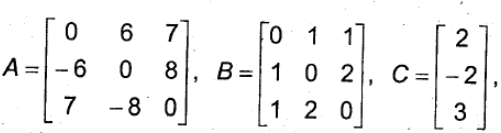calculate AC, BC and (A + B) C. Also, verify that (A + B) C = AC + BC.

Solution: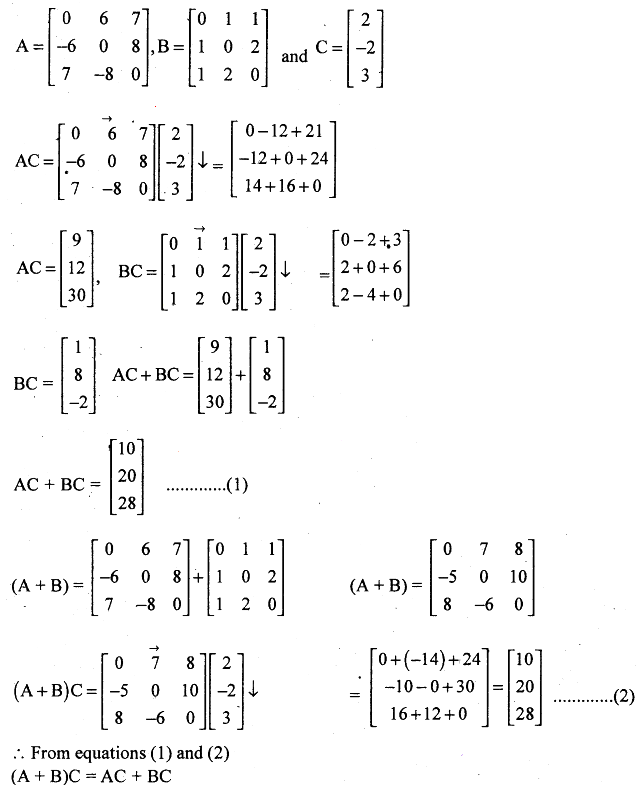Question 41: Solve the following system of linear equations by matrix method.

x – y + 2z = 7

3x + 4y – 5z = -5

2x – y + 3z = 12

Solution: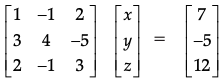AX = B

AA-1X = A-1B

IX = A-1B

X = A-1B

|A| =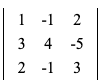= 1 · 4 · 3 + (- 1) · (- 5) · 2 + 2 · 3 · (- 1) – 2 · 4 · 2 – 1 · (- 5) · (- 1) – (- 1) · 3 · 3

= 12 + 10 – 6 – 16 – 5 + 9

= 4

Matrix of cofactors =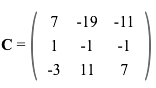Transposed matrix of cofactors = CT =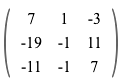A-1 = CT / |A| =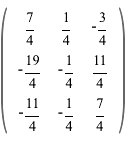X = A-1B

=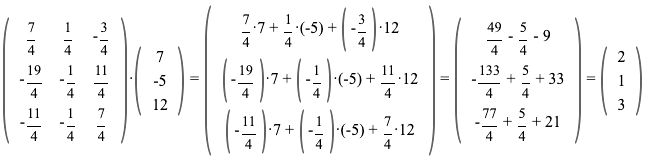x = 2, y = 1, z = 3

Question 42: If y = (tan-1 x)2, show that (x2 + 1)2 y2 + 2x (x2 + 1) y1 = 2.

Solution:

y = (tan-1 x)2

dy / dx = d (tan-1 x)2 / dx

dy / dx = 2 tan-1 x d (tan-1 x) / dx

= 2 tan-1 x * (1 / (1 + x2))

(1 / (1 + x2)) (dy / dx) = 2 tan-1 x

(1 / (1 + x2)) d2y / dx2 + (dy / dx) (0 + 2x) = 2 / (1 + x2)

(1 + x2)2 d2y / dx2 + 2x (1 + x2) (dy / dx) = 0

(x2 + 1)2 y2 + 2x (x2 + 1) y1 = 2

Question 43: Sand is pouring from a pipe at the rate of 12 cm3/s. The falling sand forms a cone on the ground in such a way that the height of the cone is always one-sixth of the radius of the base. How fast is the height of the sand cone increasing when the height is 4 cm?

Solution:

dV / dt = 12 cm3/sec

Height of the cone = (1 / 6) of the radius of the base of the cone

Volume of the cone = (1 / 3) 𝛑r2h

= (1 / 3) 𝛑 (6h)2 h [h = r / 6]

= 12𝛑h3

dV / dt = d (12𝛑h3) / dt

12 = 12𝛑 . 3h2 (dh / dt)

1 = 𝛑 * 3 (4)2 (dh / dt)

1 / 48𝛑 = dh / dt

dh / dt = 1 / 48𝛑

= 1 / (48 * (22 / 7))

= 7 / (48 * 22)

= 0.0066 cm/sec

Question 44: Find the integral of 1 / x2 + a2 with respect to x and hence find ∫[1 / x2 – 6x + 13] dx.

Solution:

I = ∫(1 / x2 + a2) dx

Let x = a tan θ

dx / dθ = d (a tan θ) / dθ = a sec2 θ

dx = a sec2 θ dθ

∫(1 / x2 + a2) dx

= ∫[1 / a2 tan2 θ + a2] a sec2 θ dθ

= ∫a sec2 θ dθ / a2 [1 + tan2 θ]

= ∫ sec2 θ dθ / a sec2 θ

= (1 / a) ∫dθ

= (1 / a) θ + c

= (1 / a) tan-1 (x / a) + c

∫[1 / x2 – 6x + 13] dx

= ∫[1 / x2 – 6x + 9 + 4] dx

= ∫[1 / (x – 3)2 + 22] dx

= (1 / 2) tan-1 |(x – 3) / 2| + c

Question 45: Using integration, find the area of the region bounded by a triangle whose vertices are (1, 0), (2, 2) and (3, 1).

Solution: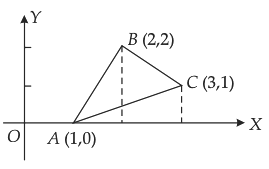Area of the region = Area of triangle ABD + Area of trapezium BDEC – Area of triangle AEC

y = 2 (x – 1), y = 4 – x, y = (1 / 2) (x – 1)

Area of triangle ABC = ∫12 2 (x – 1) dx + ∫32 (4 – x) dx – ∫13 (x – 1) / 2 dx

= 2 [x2 / 2 – x]12 + [4x – (x2 / 2)]32 – (1 / 2) [x2 / 2 – x]13

= 2 [(2 – 2) – ((1 / 2) – 1)] + [(4 * 3 – (9 / 2)] – (1 / 2) [((9 / 2) – 3) – ((1 / 2) – 1)]

= 3 / 2

Question 46: Find the general solution of the differential equation x (dy / dx) + 2y = x2 logx.

Solution:

x (dy / dx) + 2y = x2 logx

(dy / dx) + (2 / x) y = x log x

This is of the form (dy / dx) + Py = Q.

P = 2 / x

Q = x log x

IF = e∫P dx

= e∫2 log x dx

= x2

The solution to the differential equation is

y * IF = ∫IF * Q dx + c

y * x2 = ∫x2 * x log x dx + c

x2 y = ∫x3 log x dx + c

x2 y = log x ∫x3 dx – ∫[d (logx) / dx ∫x3 dx] dx + c

x2y = (x4 / 4) logx – ∫(1 / x) * (x4 / 4) dx + c

y = (x2 / 4) logx – (x2 / 16) + c / x2

16y = 4x2 logx – x2 + 16cx-2

Question 47: Derive the equation of the line in space passing through two given points, both in vector and Cartesian form.

Solution: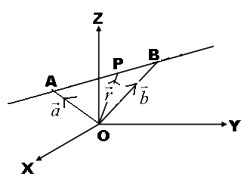Let a, b and r be the position vectors of the two points A (x1, y1, z1) is (x2, y2, z2) and p (x, y, z) respectively.

AP = OP – OA = r – a

AB = OB – OA = b – a

The point p will lie on the line AB if and only if AP and AB are collinear.

AP = ƛ AB

(r – a) = ƛ (b – a)

r = a + ƛ (b – a) is the vector equation of the line passing through two points.

Let r = xi + yj + zk, a = x1i + y1j + z1k, b = x2i + y2j + z2k, r = a + ƛ (b – a)

xi + yj + zk = x1i + y1j + z1k + ƛ ((x2 – x1) i + (y2 – y1) j + (z2 – z1) k)

= [x1 + ƛ (x2 – x1)] i + [y1 + ƛ (y2 – y1)] j + [z1 + ƛ (z2 – z1)] k

x = x1 + ƛ (x2 – x1)

x – x1 = ƛ (x2 – x1)

ƛ = (x – x1) / (x2 – x1)

y = y1 + ƛ (y2 – y1)

y – y1 = ƛ (y2 – y1)

ƛ = (y – y1) / (y2 – y1)

z = z1 + ƛ (z2 – z1)

z – z1 = ƛ (z2 – z1)

ƛ = (z – z1) / (z2 – z1)

Hence the equation of the line passing through the points (x1, y1, z1) and (x2, y2, z2) is (x – x1) / (x2 – x1) = (y – y1) / (y2 – y1) = (z – z1) / (z2 – z1).

Question 48: If a fair coin is tossed 10 times, find the probability of

Solution:

Let X denote the number of heads in an experiment of 10 trials.

n = 10

p = 1 / 2

q = 1 – p = 1 – (1 / 2) = 1 / 2

P (X = r) = nCr pr qn-r

[i] P (X = 6)

= 10C6 (1 / 2)6 (1 / 2)10-6

= 10C6 (1 / 2)10

= 105 / 512

[ii] P (at least 6 heads)

= P (X ≥ 6)

= P (X = 6) + P (X = 7) + P (X = 8) + P (X = 9) + P (X = 10)

= 10C6 (1 / 2)10 + 10C7 (1 / 2)10 + 10C8 (1 / 2)10 + 10C9 (1 / 2)10 + 10C10 (1 / 2)10

= 193 / 512

PART – E

Answer any one of the following questions. [1 * 10 = 10]

Question 49: [a] Prove that ∫0a f (x) dx = ∫0a f (a – x) dx and hence evaluate ∫0a √x / (√x + √a – x) dx.

[b] Prove that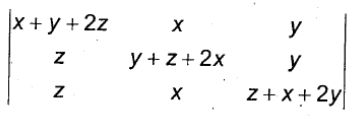= 2 (x + y + z)3.

Solution:

[a] ∫0a f (x) dx = ∫0a f (a – x) dx

Let a – x = t

x = a – t

d (a – x) / dx = dt / dx

-1 = dt / dx

dx = -dt

When x = 0, a = t and x = a, t = 0

ab f (x) dx = – ∫0a f (a – t) dt

ab f (x) dx = ∫0a f (a – t) dt

ab f (x) dx = ∫0a f (a – x) dx

I = ∫0a √x / (√x + √a – x) dx

= ∫0a √a – x / (√a – x + √a – a + x) dx

2I = ∫0a √x / (√x + √a – x) dx + ∫0a √a – x / (√x + √a – x) dx

= ∫0a (√x + √a – x) / (√x + √a – x) dx

= ∫0a dx

= [x]0a

= a – 0

2I = a

I = a / 2

[b]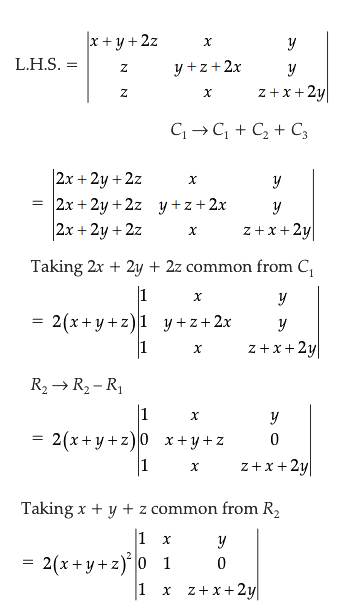Expand along R2

= 2 (x + y + z)2 (z + x + 2y – y)

= 2 (x + y + z)2 (x + y + z)

= 2 (x + y + z)3

= R.H.S.

Question 50: [a] Solve the following problem graphically:

Minimise and Maximize:

Z = 3x + 9y

Subject to constraints

x + 3y ≤ 60

x + y ≥ 10

x ≤ y

x ≥ 0, y ≥ 0

[b] Find the relationship between a and b so that the function f defined by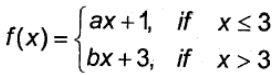is continuous at x = 3.

Solution:

[a]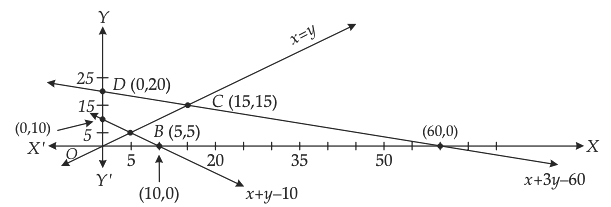The corner points are A (0, 10), B (5, 5), C (15, 15) and D (0, 20).

 Points Z A (0, 10) 90 B (5, 5) 60 ← Minimum C (15, 15) 180 ← Maximum D (0, 20) 180 ← Maximum

The minimum value of Z = 60 at point B (5, 5).

The maximum value of Z = 180 at the points D (0, 20) and C (15, 15).

[b]f (x) is continuous at x = 3.

lim x→3+ f (x) = lim x→3- f (x) = f (3)

lim x→3+ f (x) = lim x→3+ f (bx + 3)

= lim h→0 [b (3 + h) + 3]

= b (3 + 0) + 3

= 3b + 3

lim x→3- f (x) = 3b + 3

lim x→3- f (x) = lim x→3- (ax + 1)

= lim h→0 [a (3 – h) + 1]

lim x→3- f (x) = a (3 – 0) +1

= 3a + 1

lim x→3+ f (x) = lim x→3- f (x)

3b + 3 = 3a + 1

3 – 1 = 3a – 3b

2 = 3 (a – b)

∴ a – b = 2 / 3